# Selina Solutions Concise Physics Class 10 Chapter 2 Work, Energy and Power

Selina Solutions Concise Physics Class 10 Chapter 2 Work, Energy and Power are available for students in lucid language for better understanding. These solutions are very useful for students to examine their capacity while solving textbook questions. Selina solutions are designed by our subject experts in order to help students in exam preparation.

ICSE Class 10 Physics Selina Solutions improves the problem-solving abilities among students. By practising these solutions, students will be able to boost their exam preparation. Concise Selina Solutions for Class 10 Physics will help students to understand complex topics easily. These solutions promote an in-depth understanding of difficult questions. Concise Selina Solutions for Class 10 Physics provide students with an extra edge in exam preparation. Selina Solutions can be downloaded with free PDF option from the links provided here

## Download PDF of Selina Solutions Concise Physics Class 10 Chapter 2 Work, Energy and Power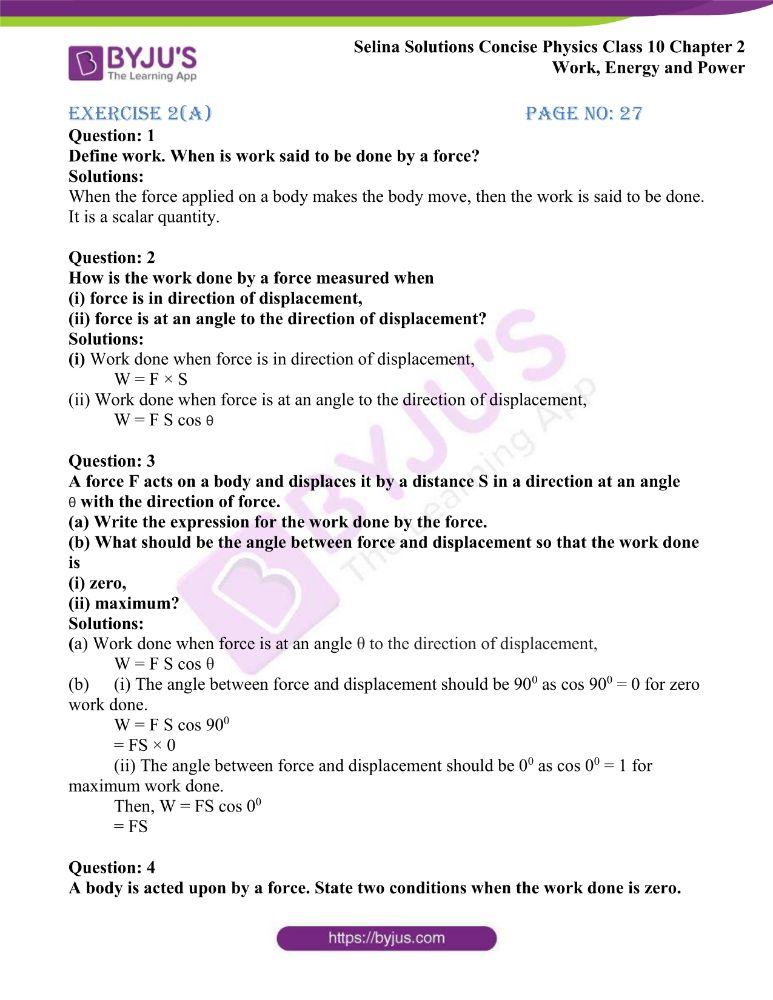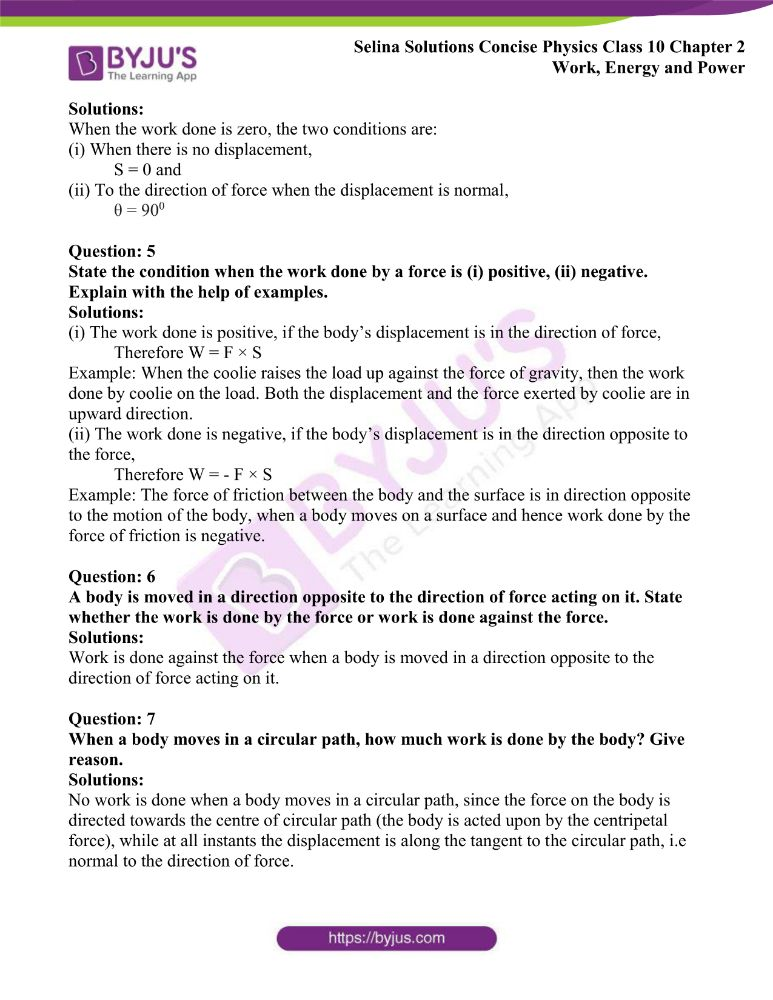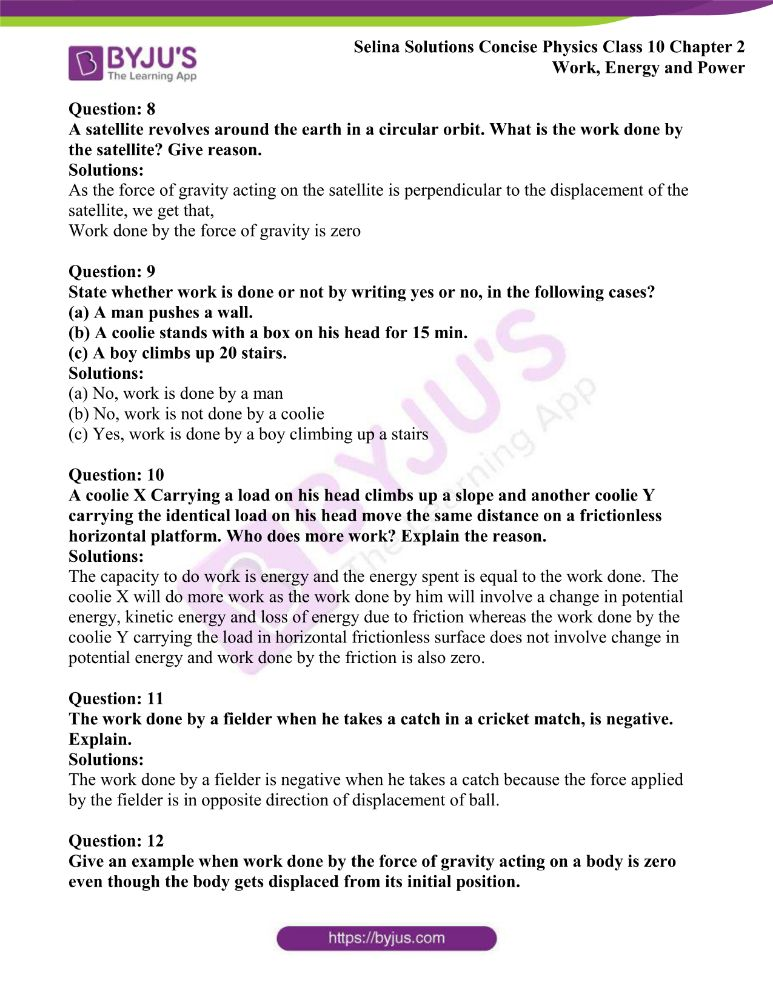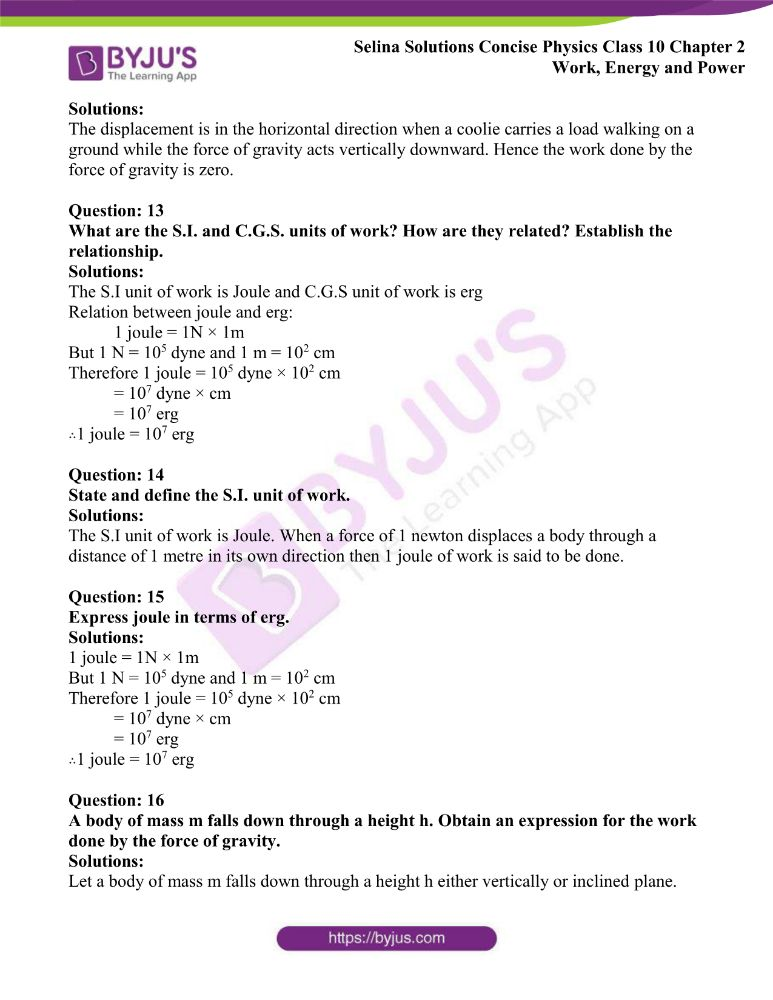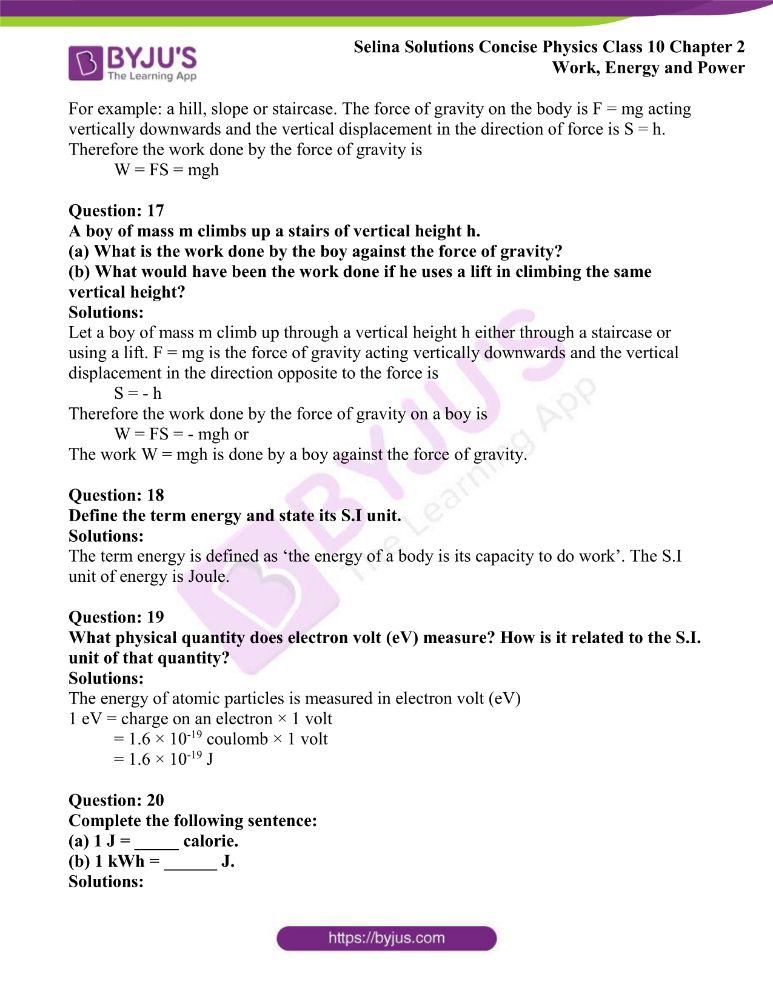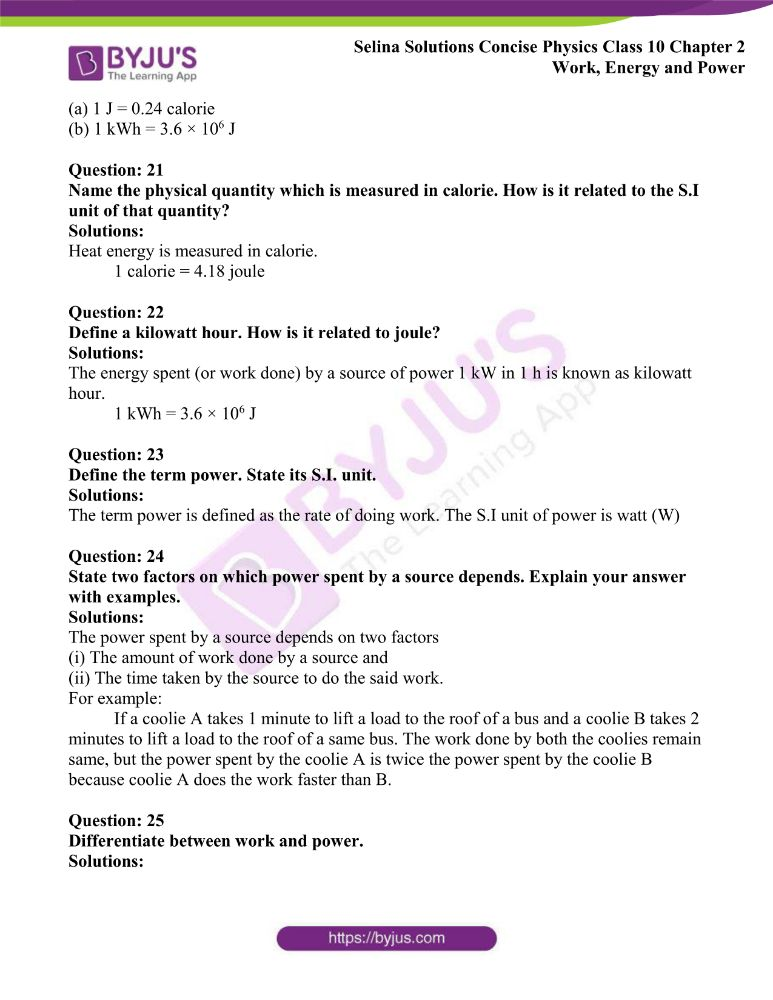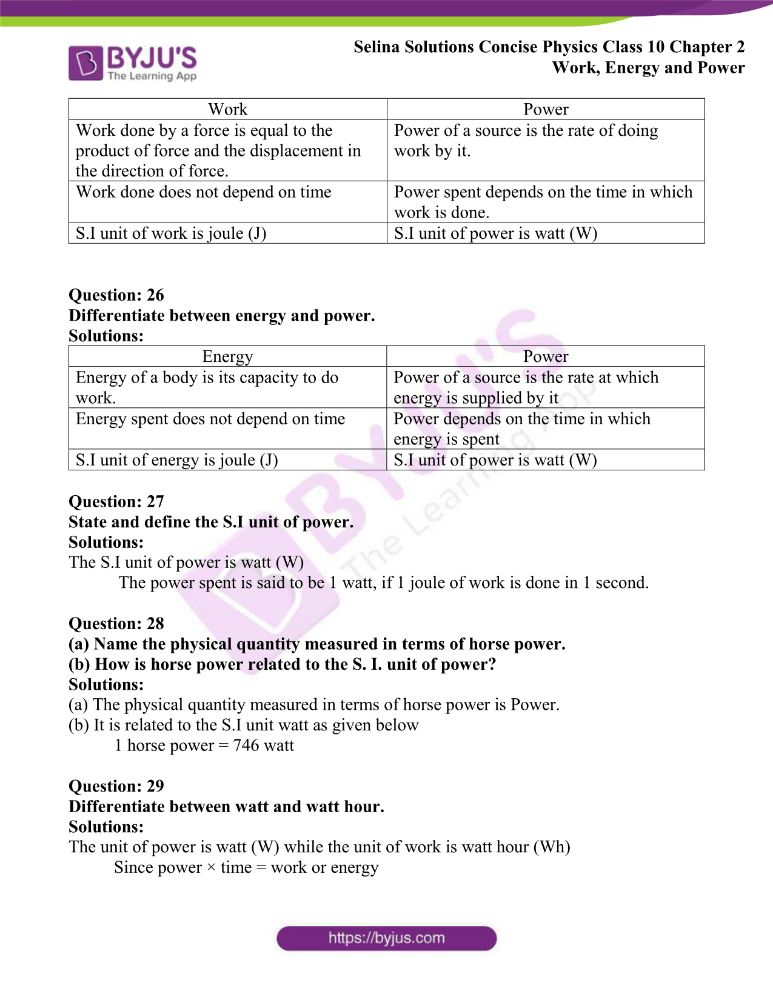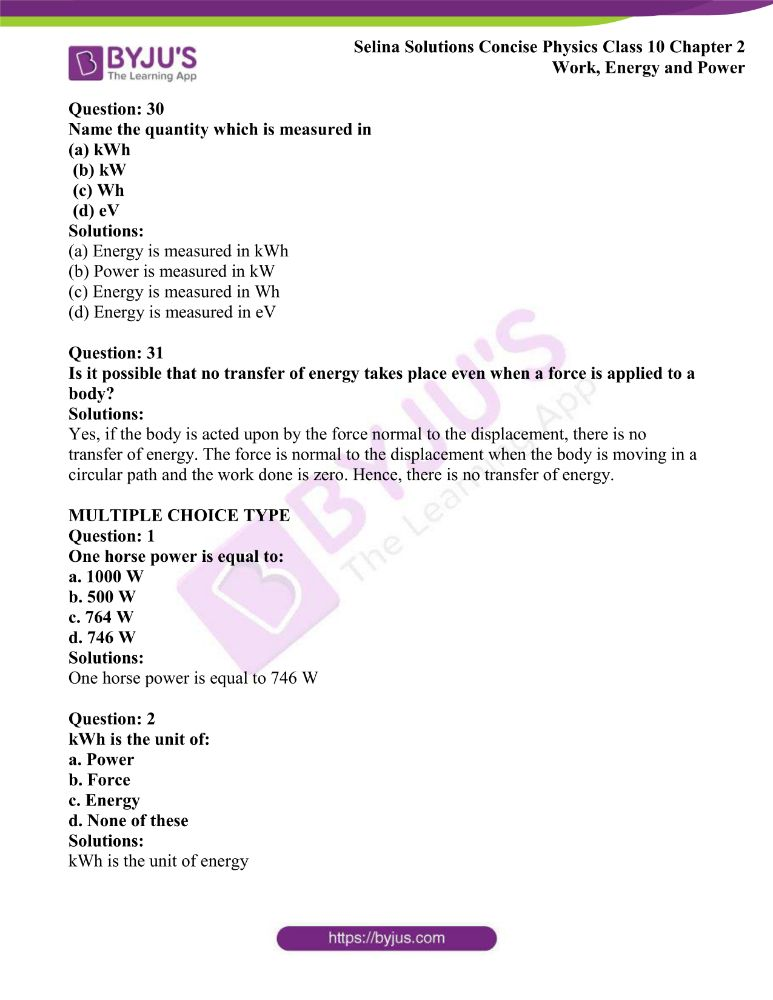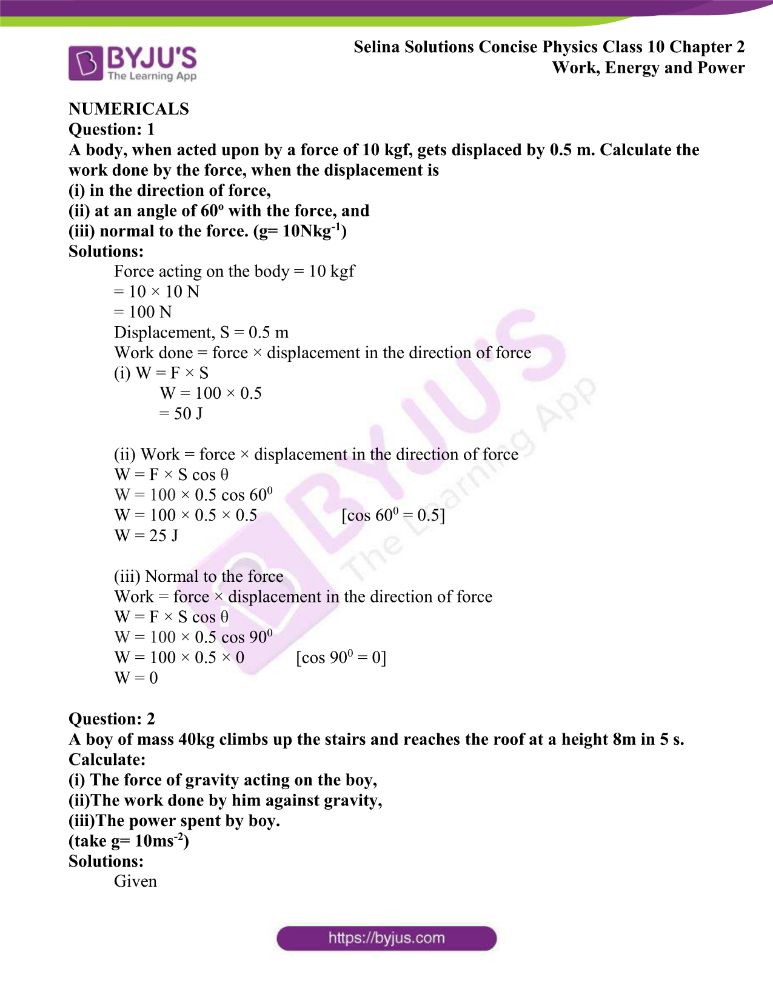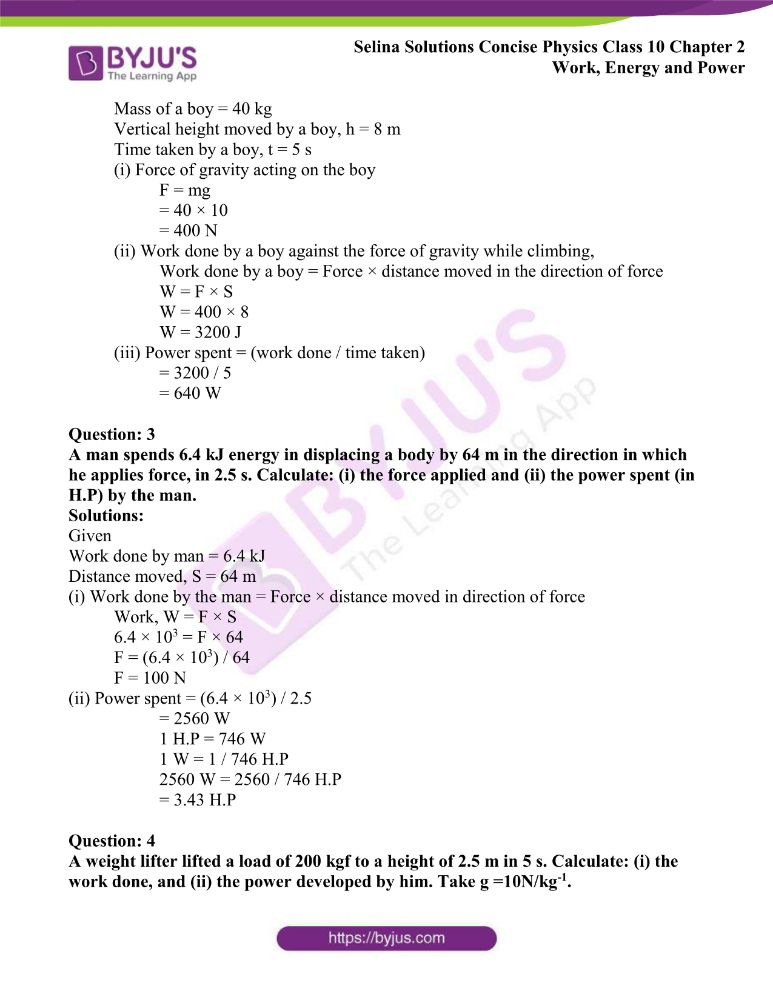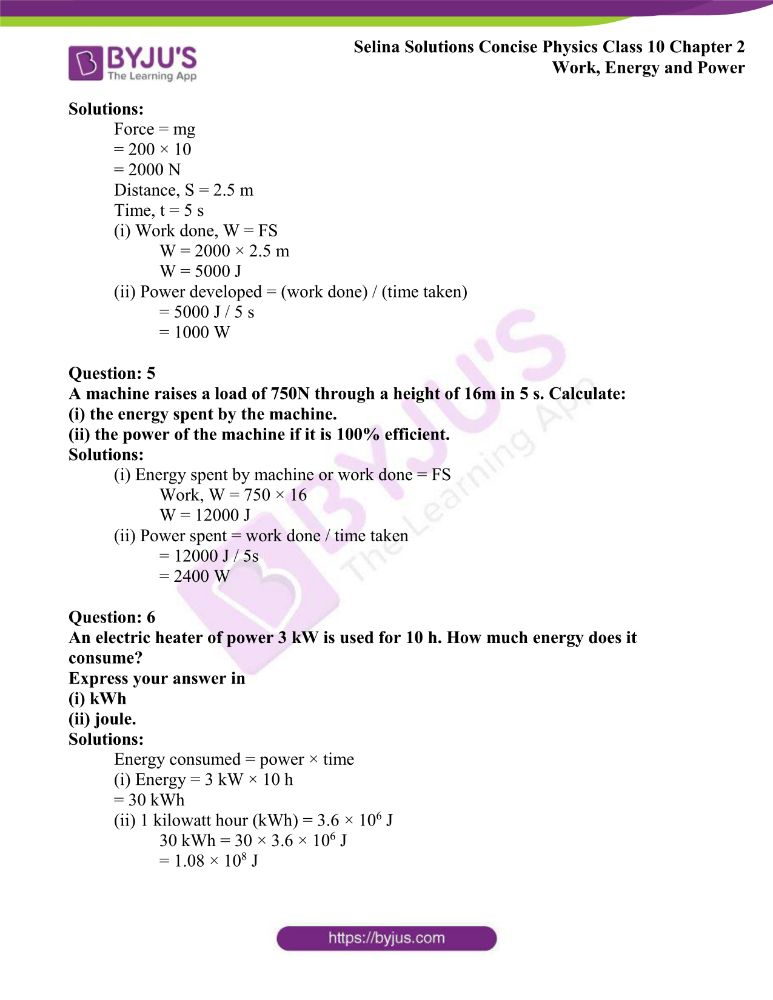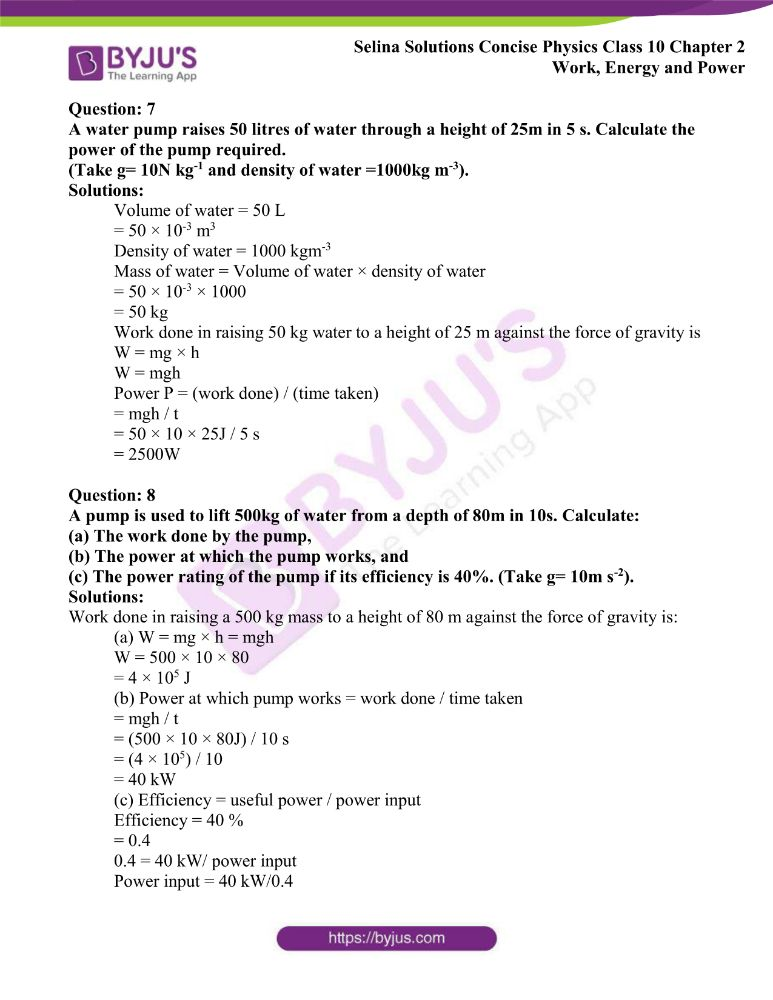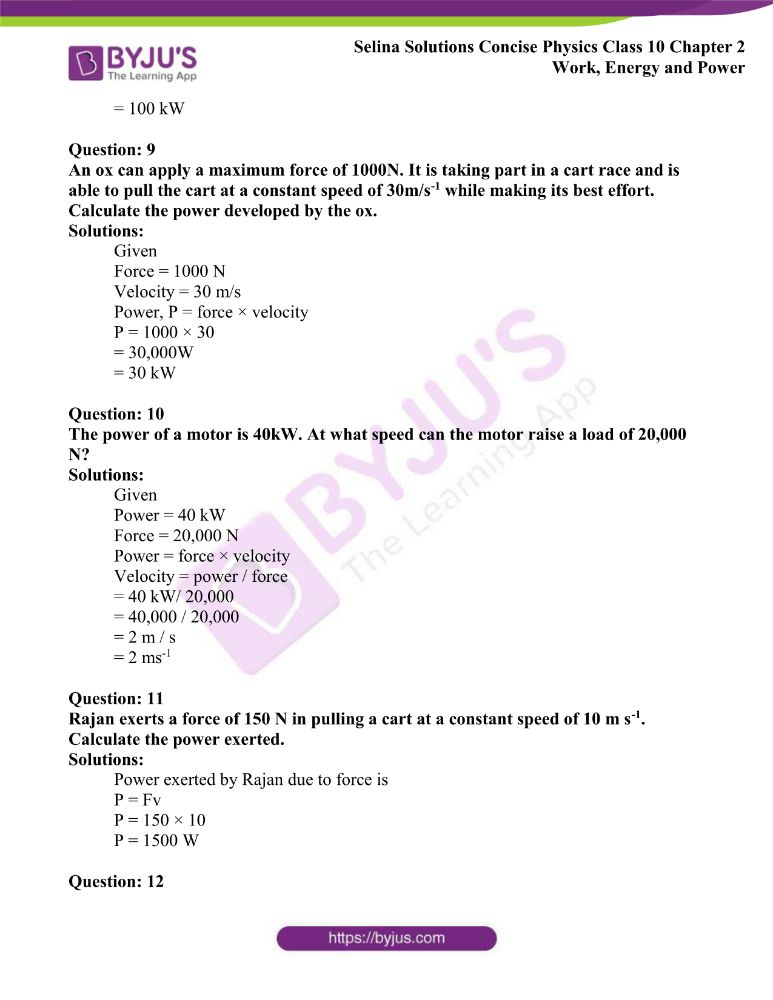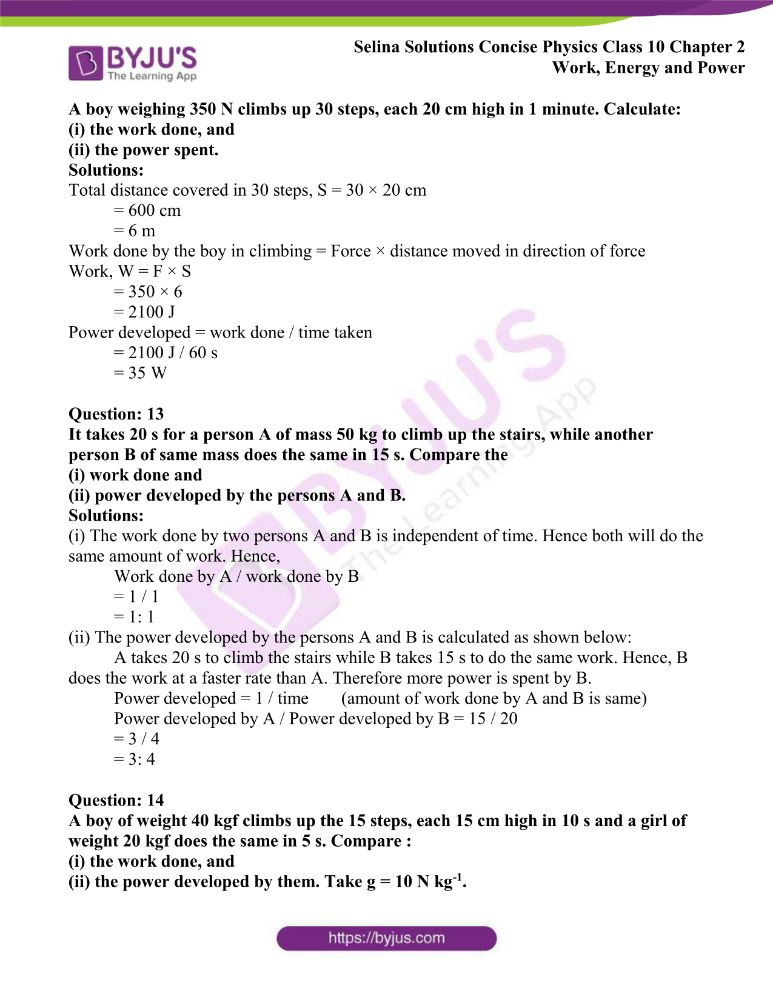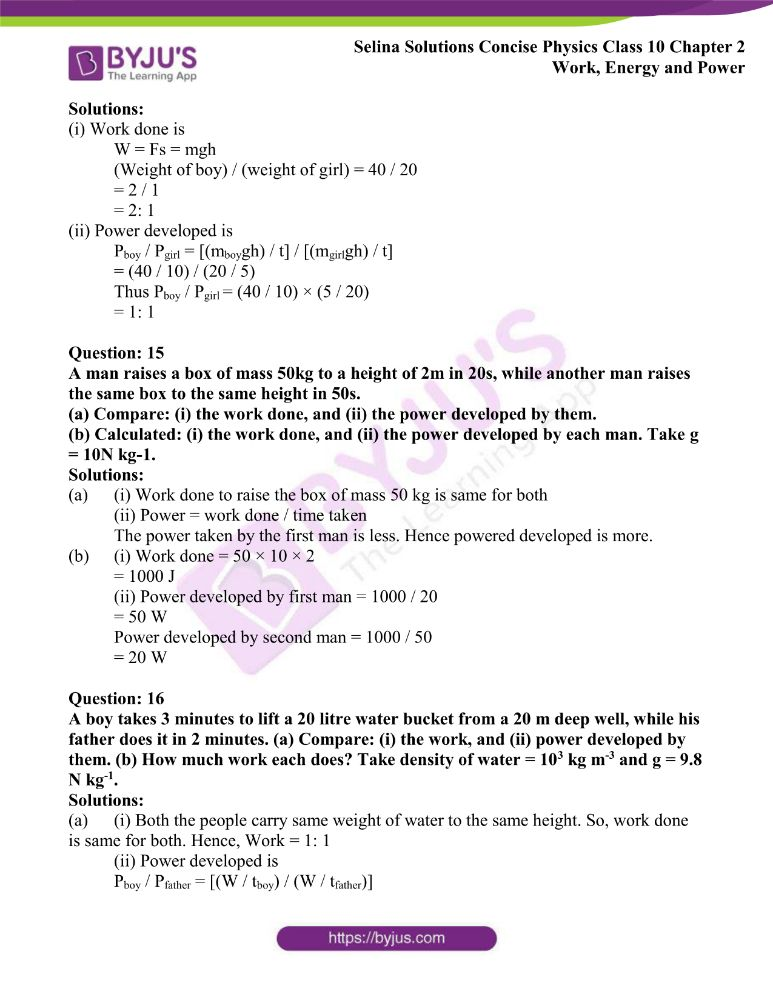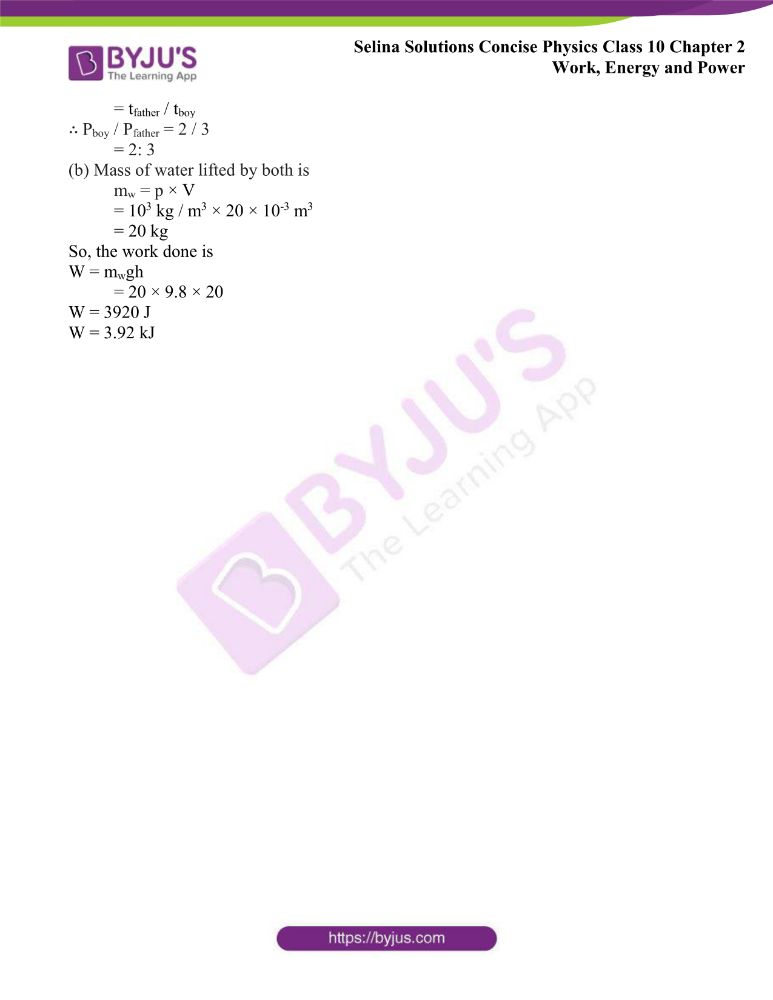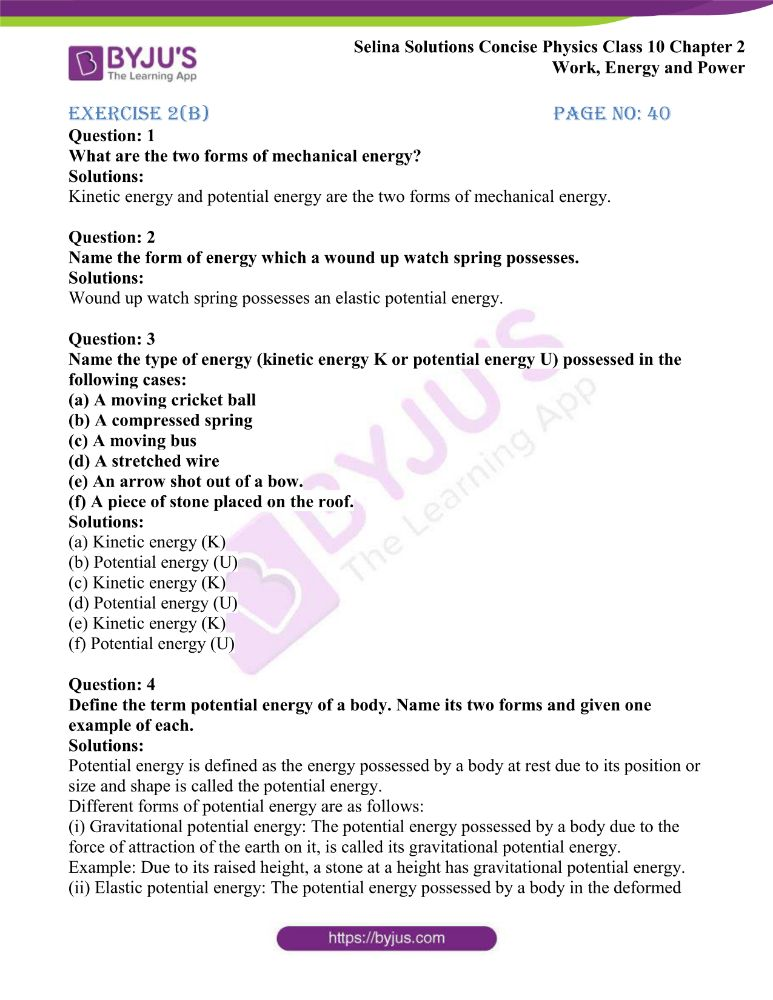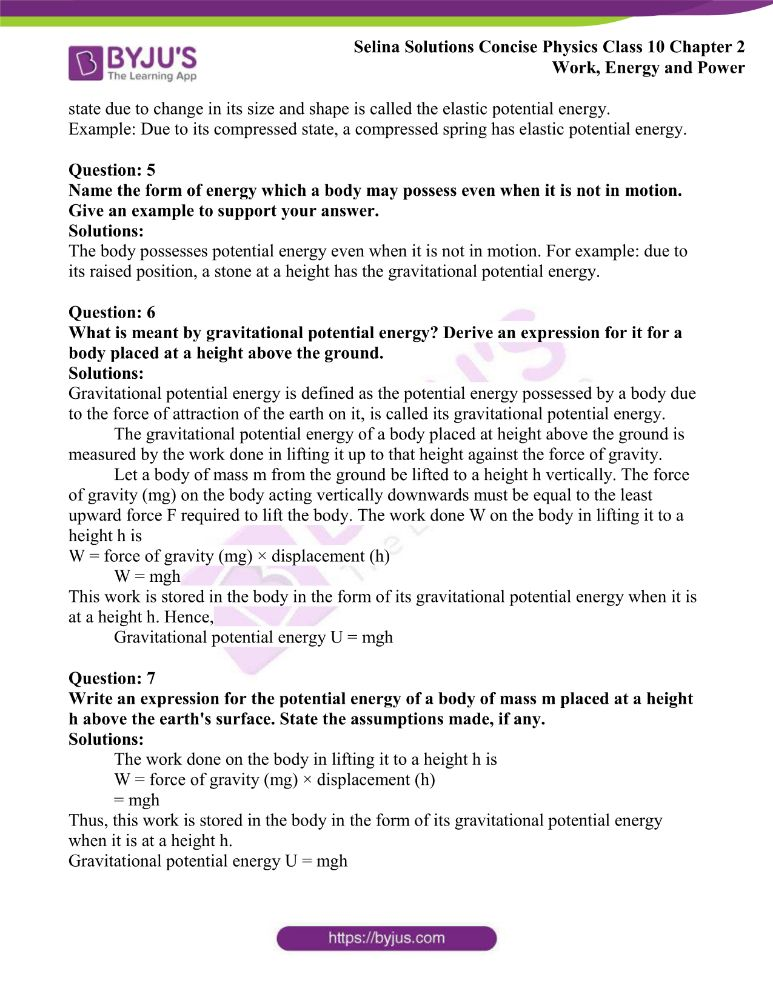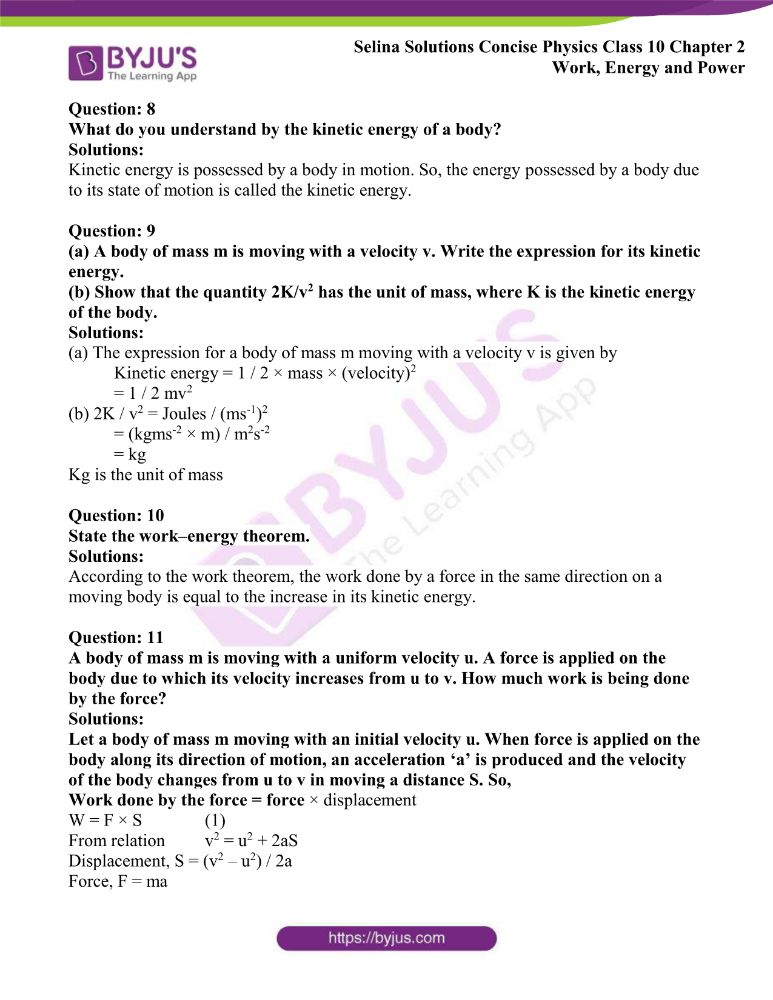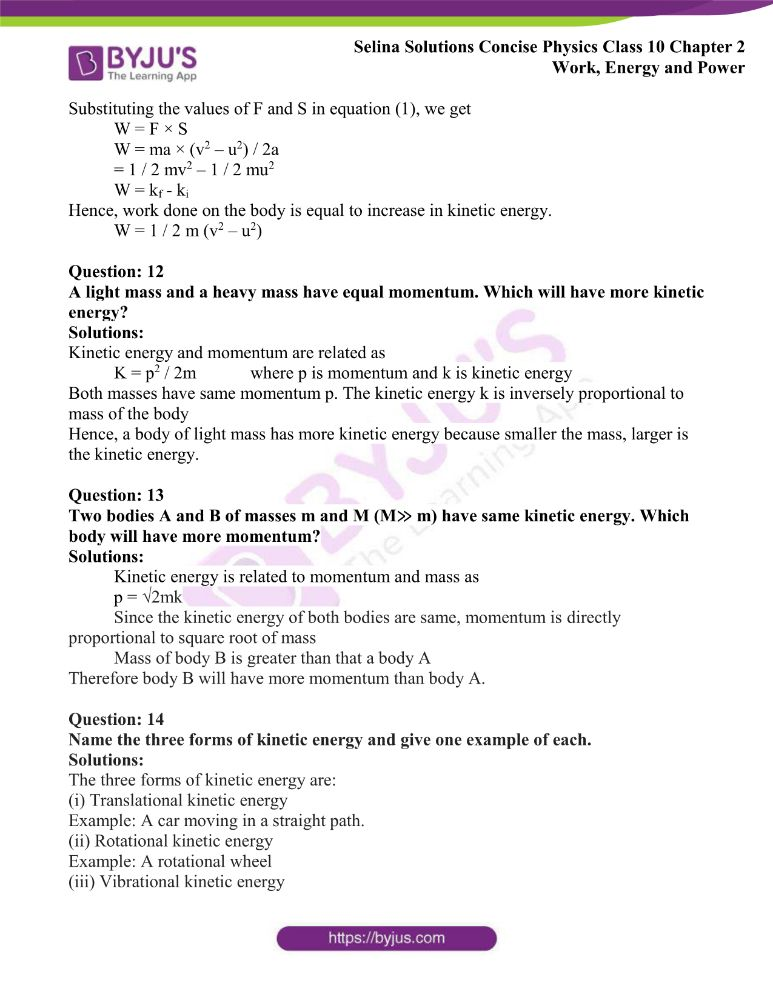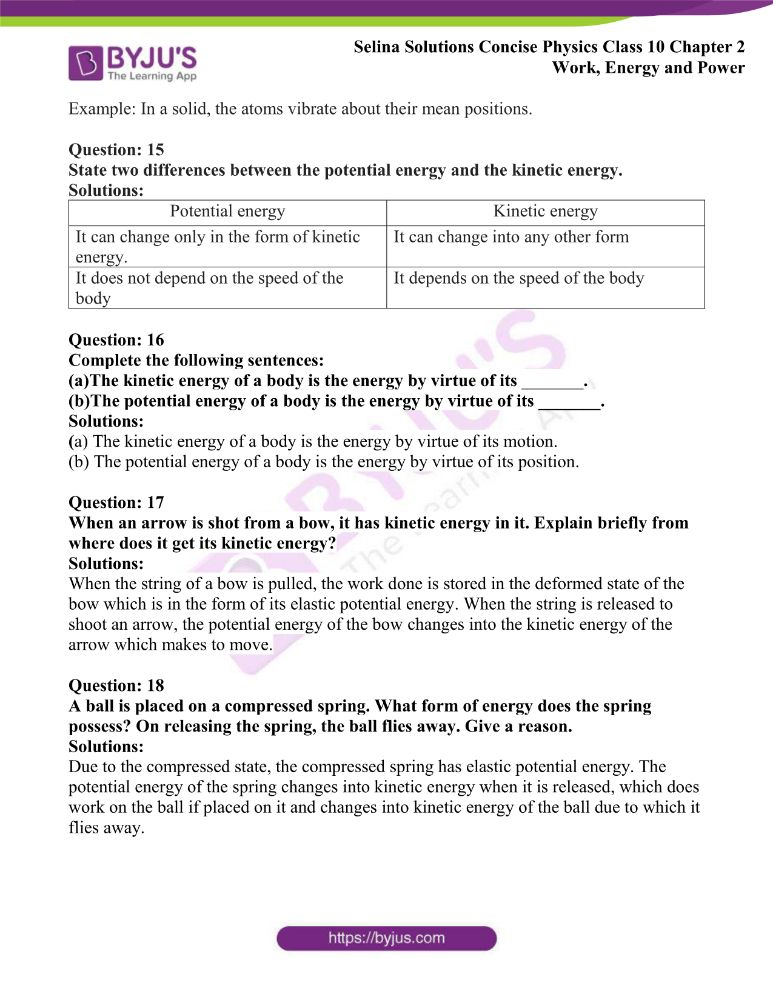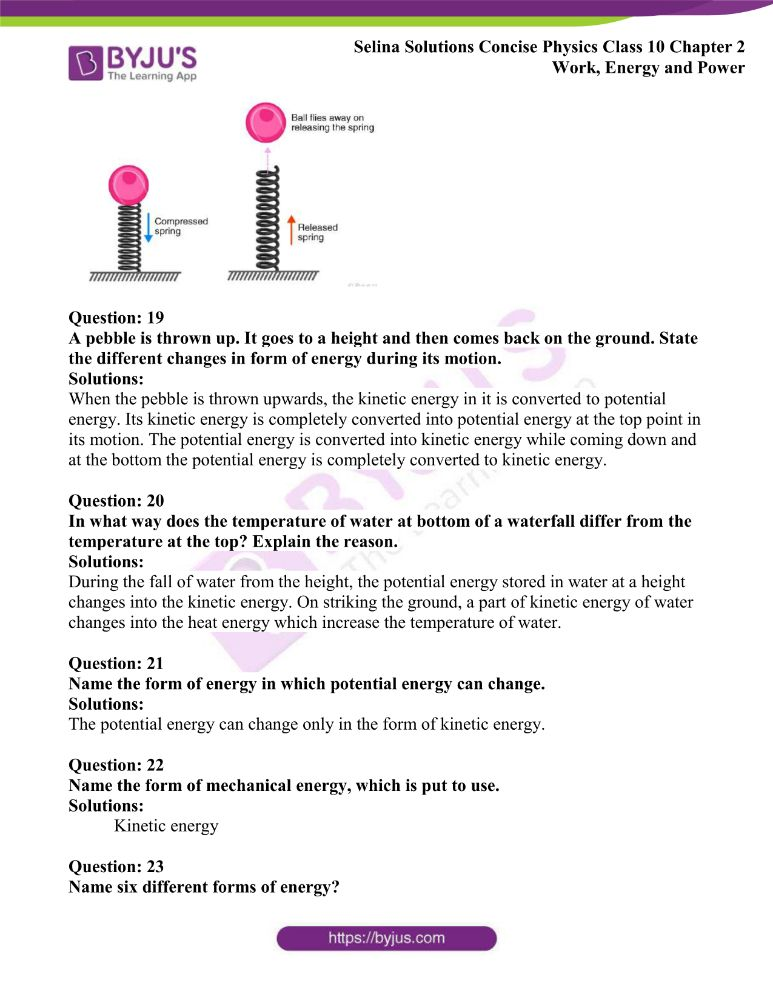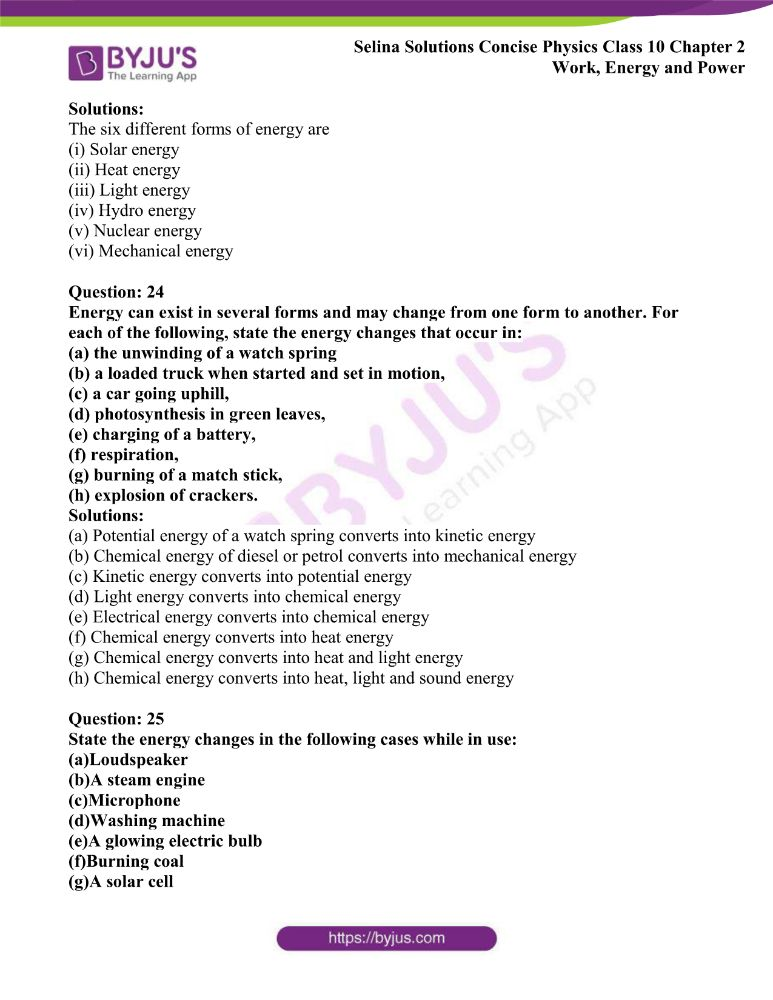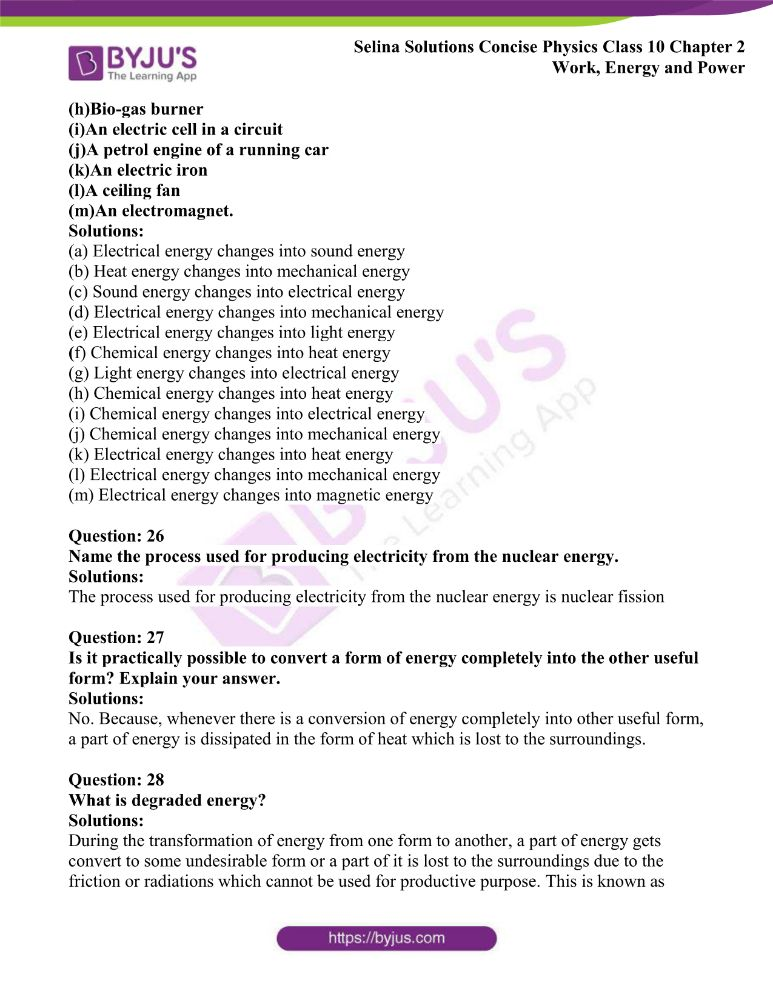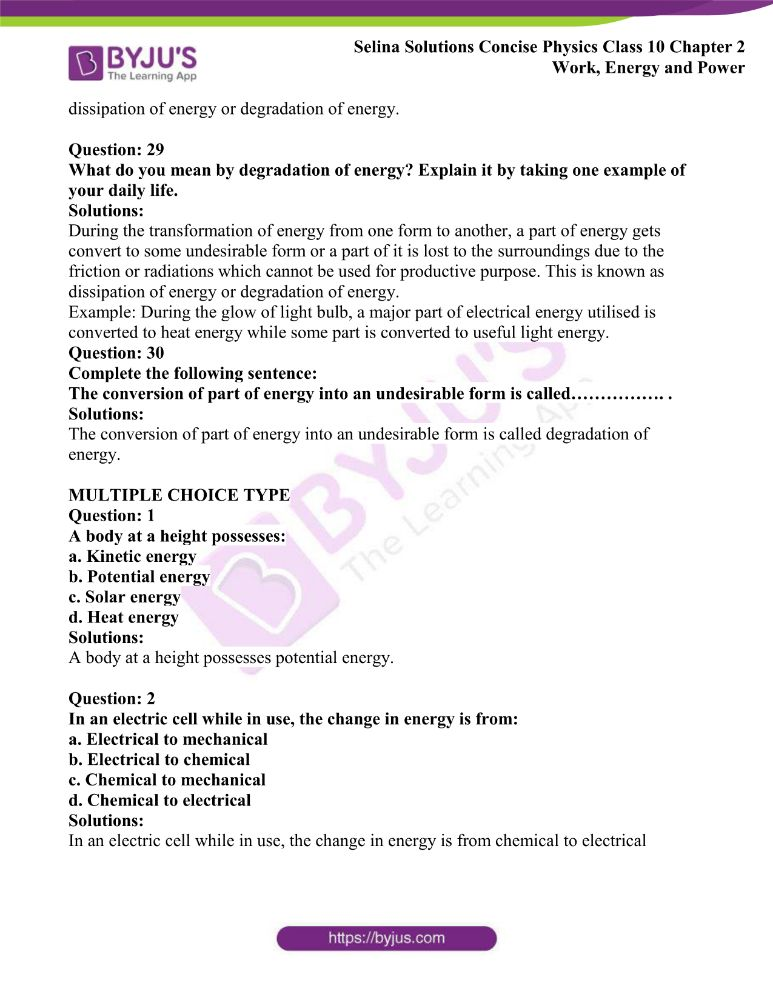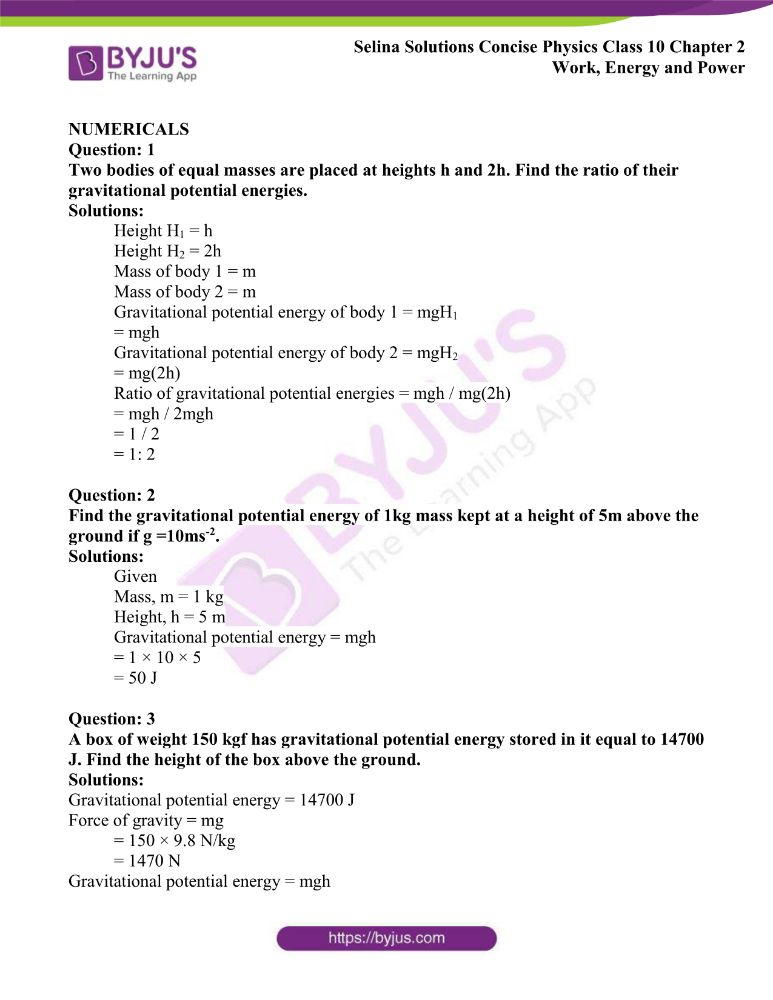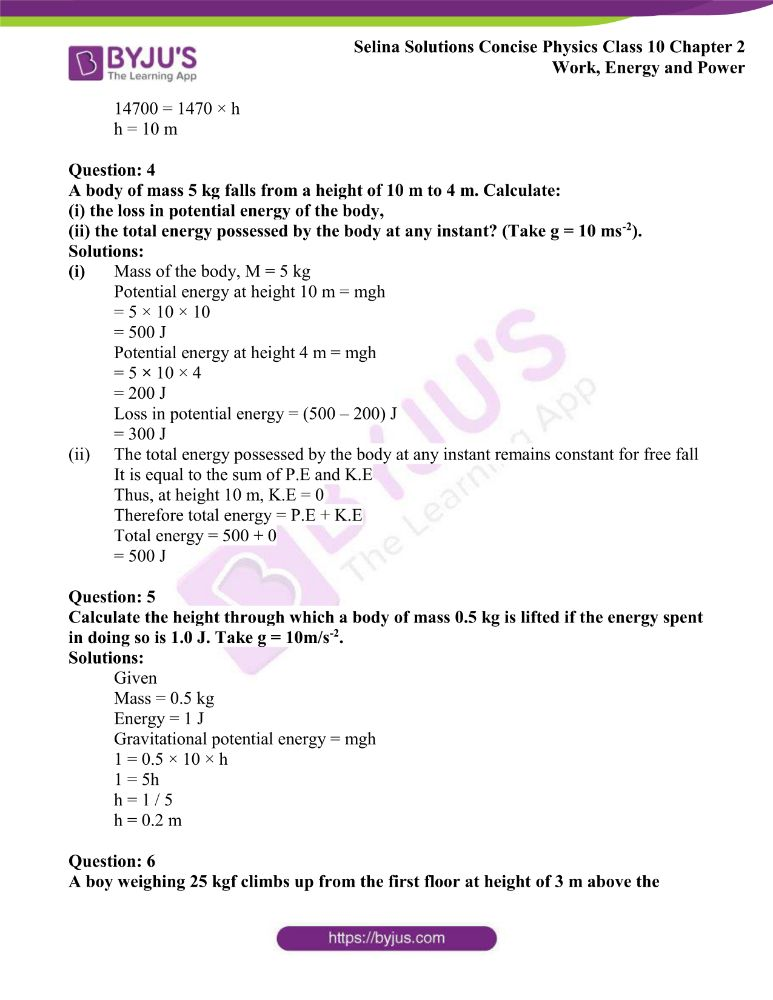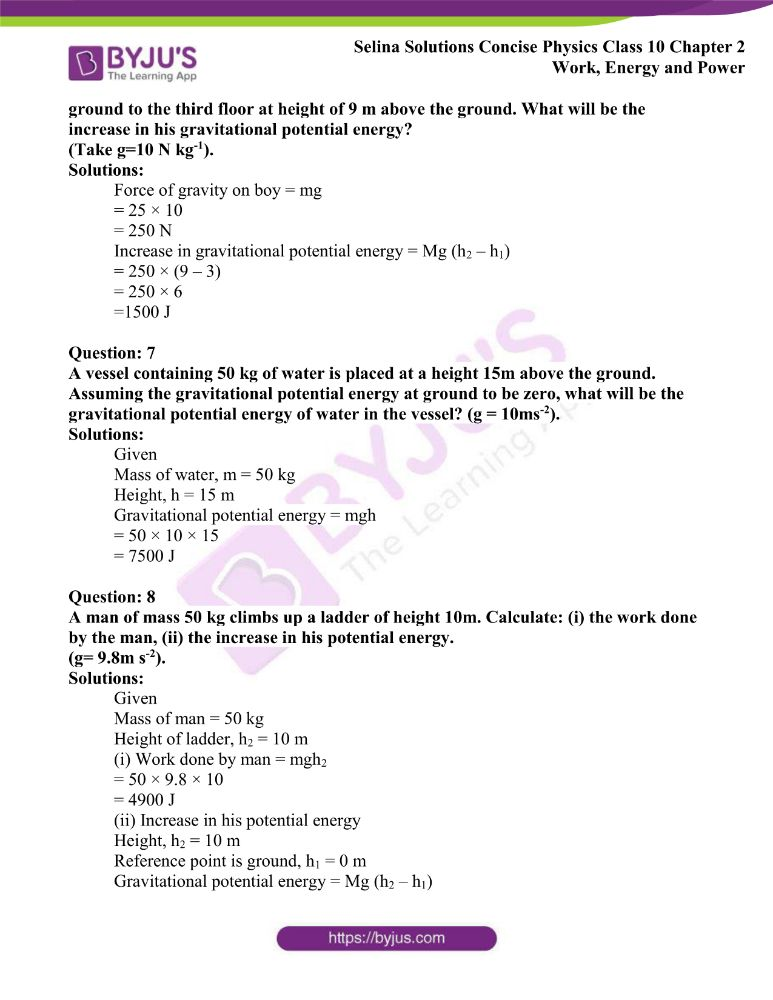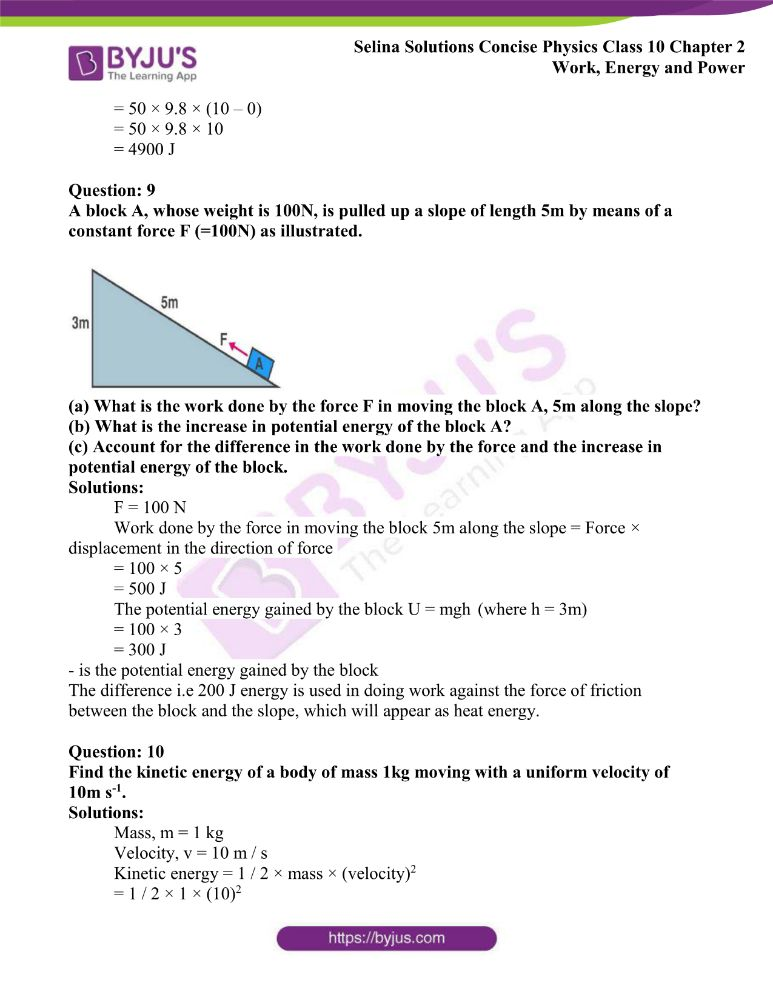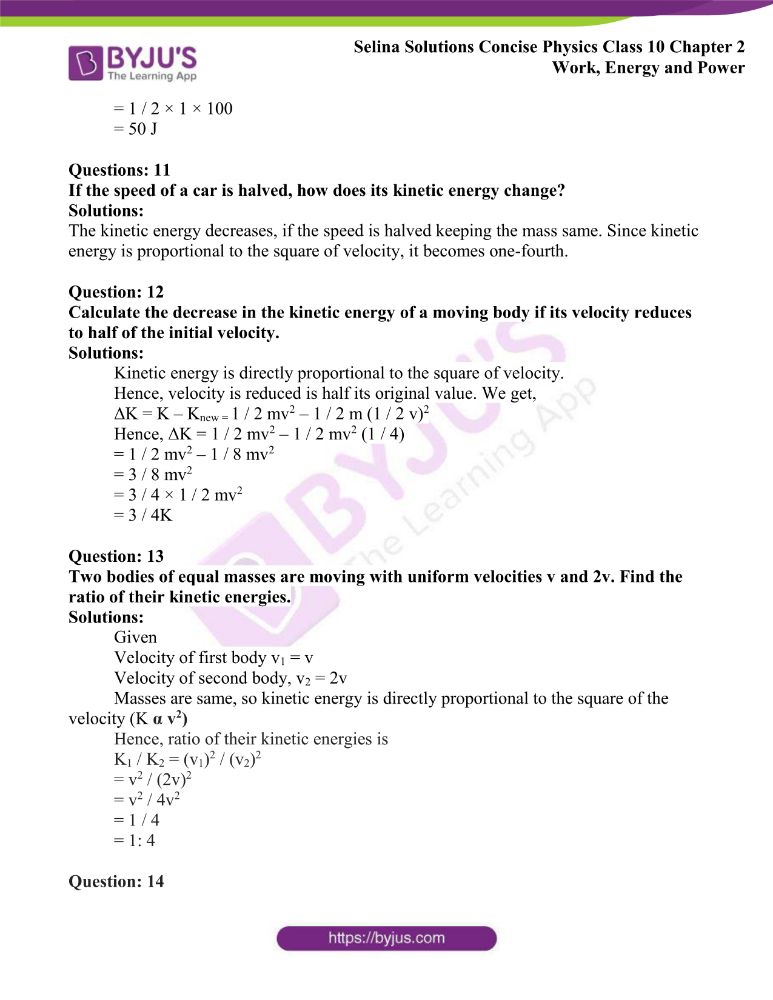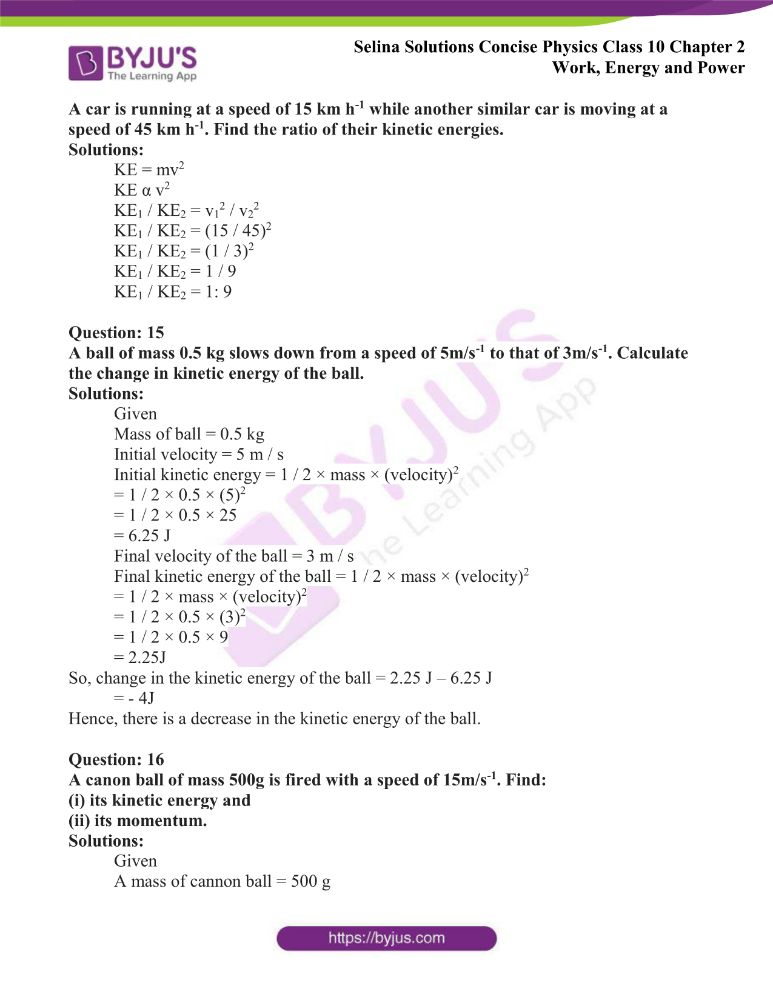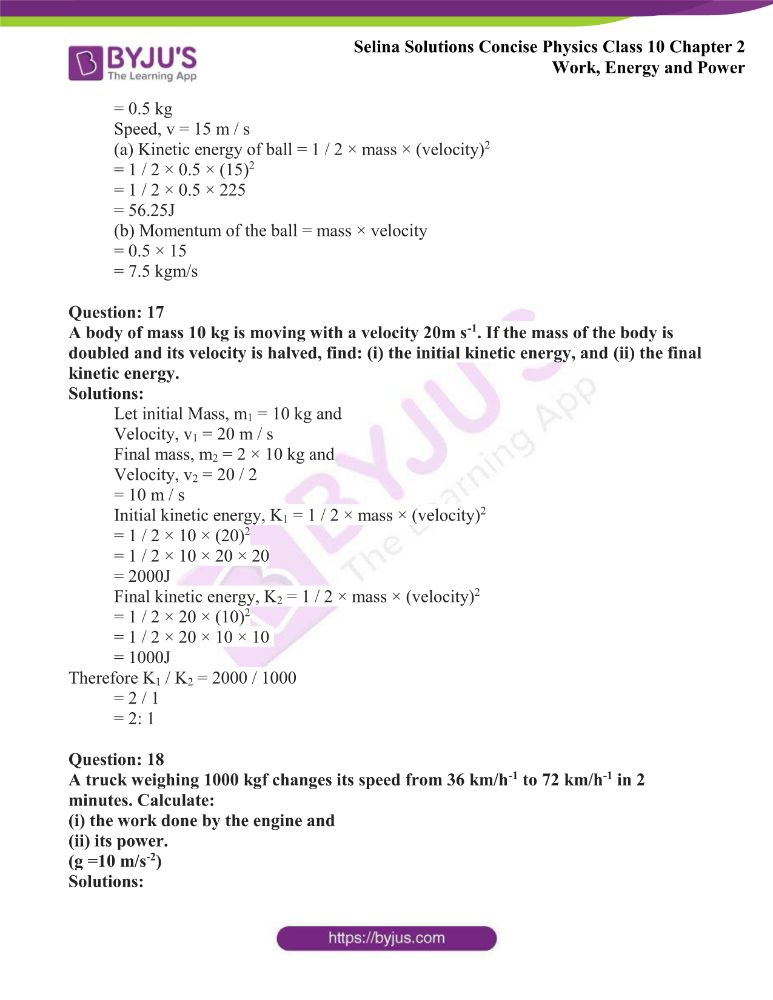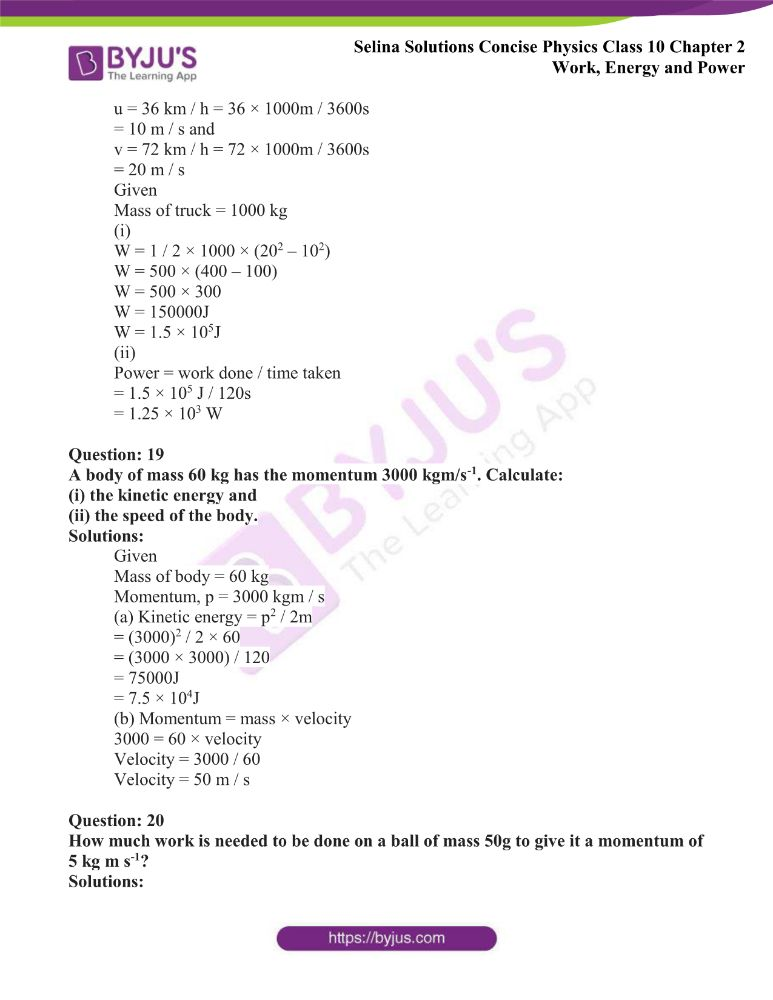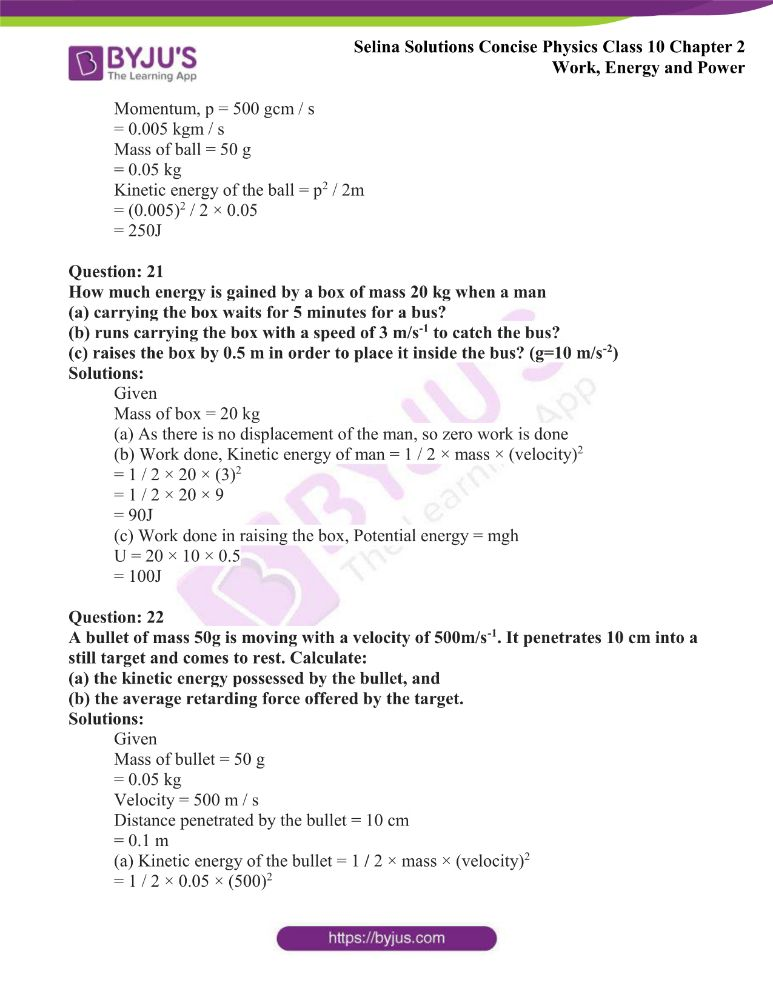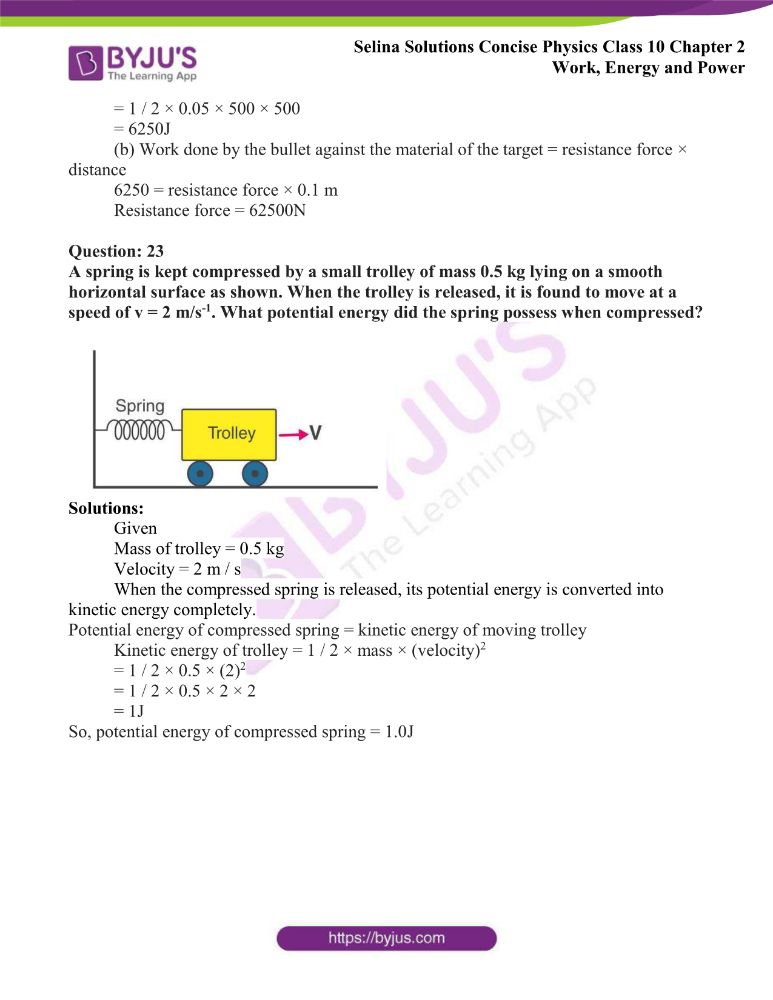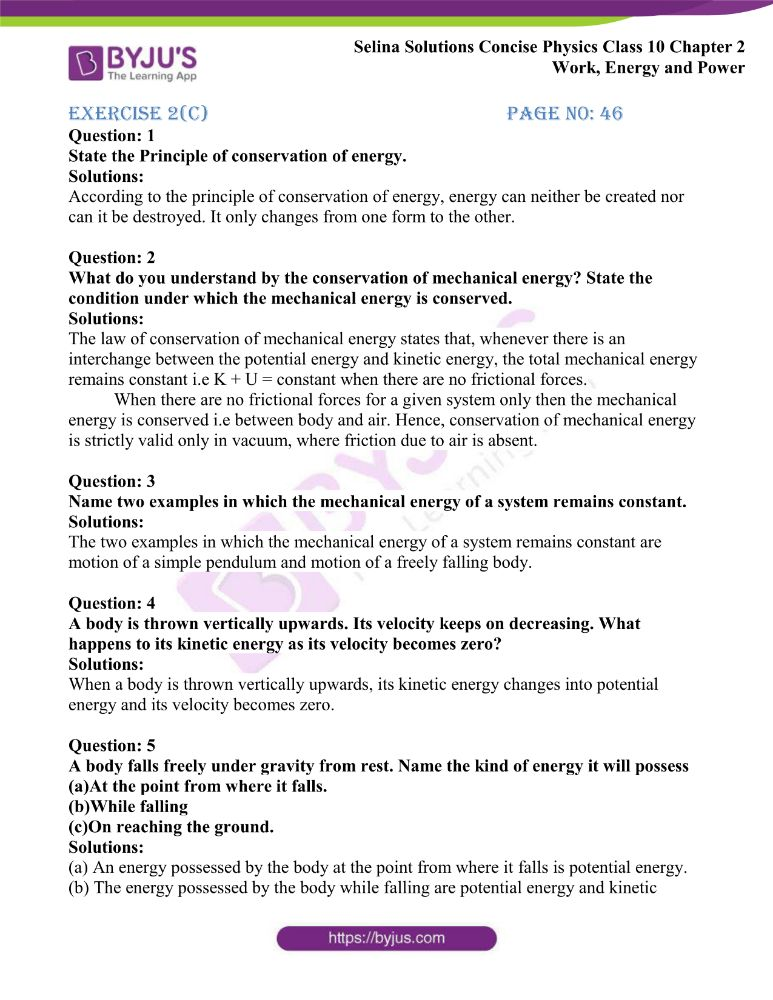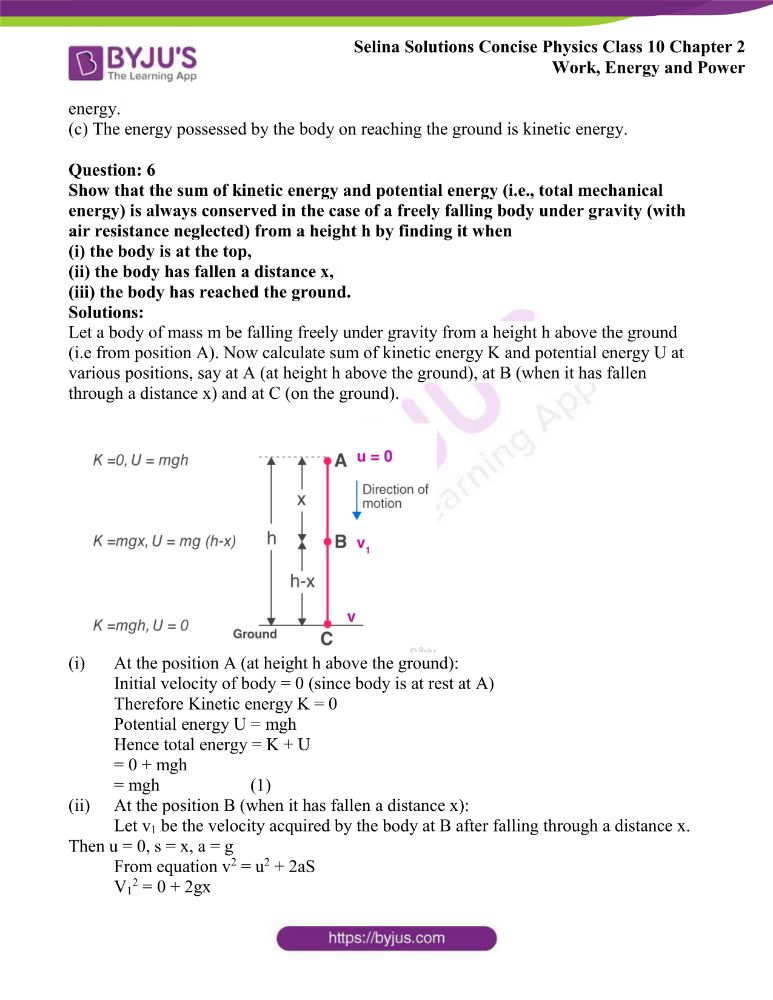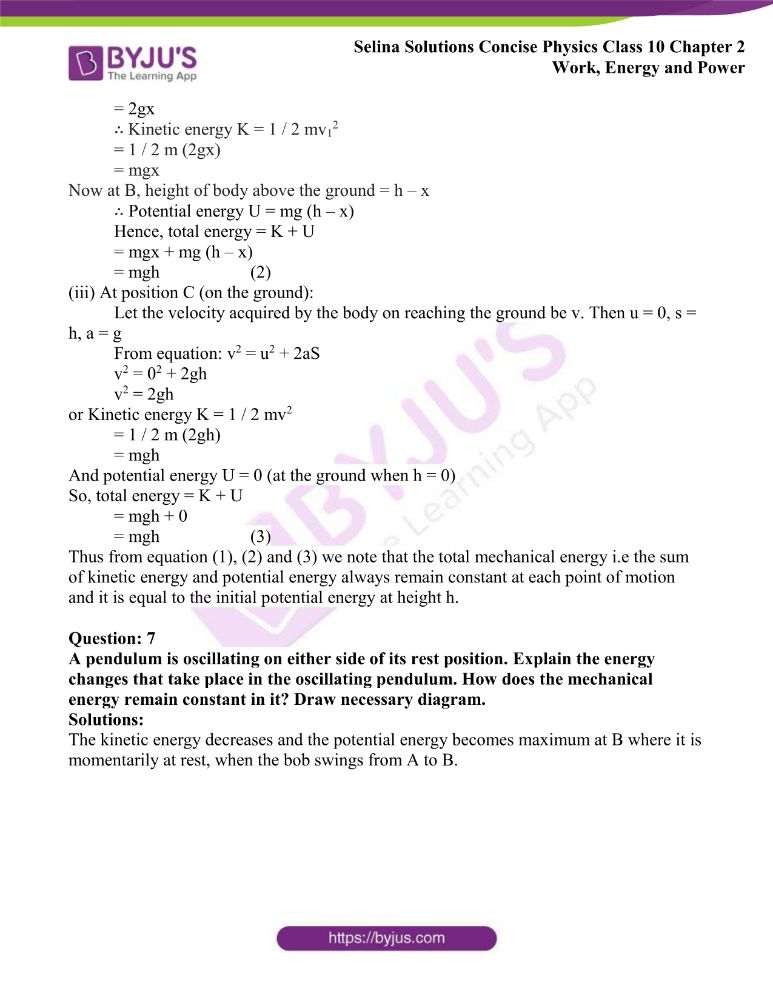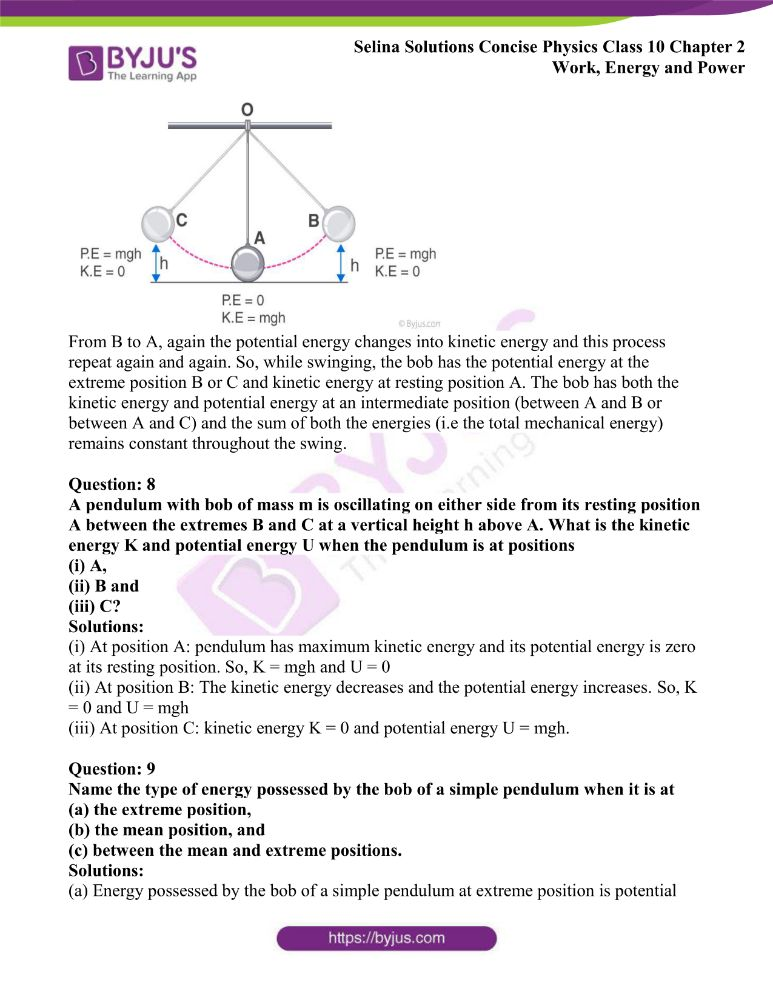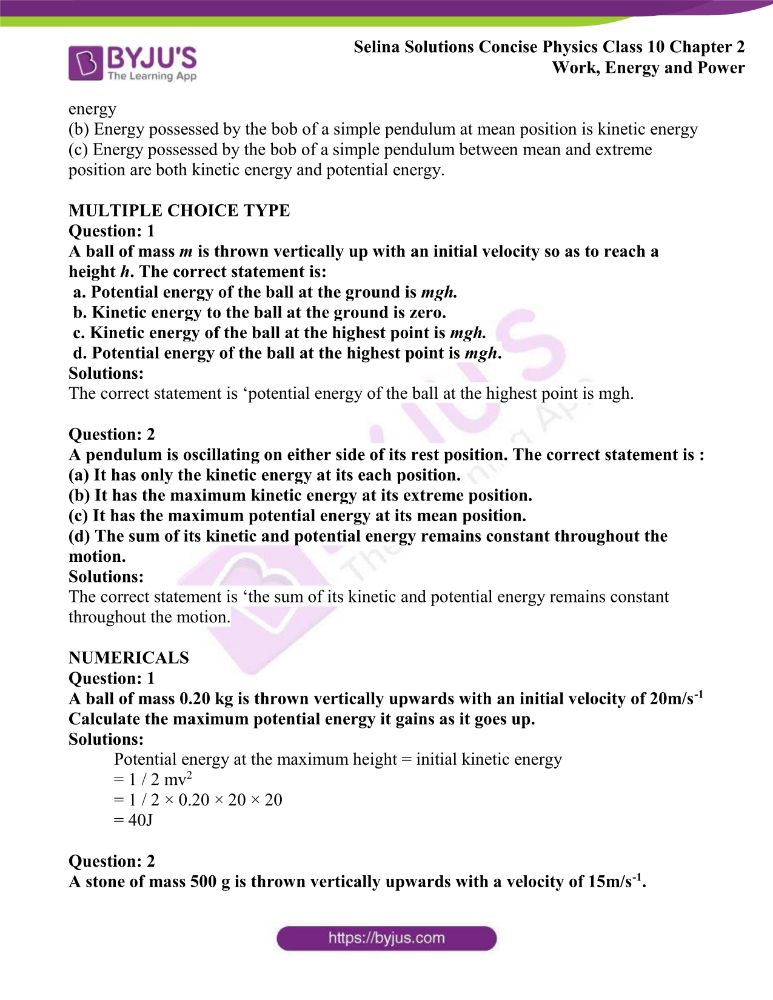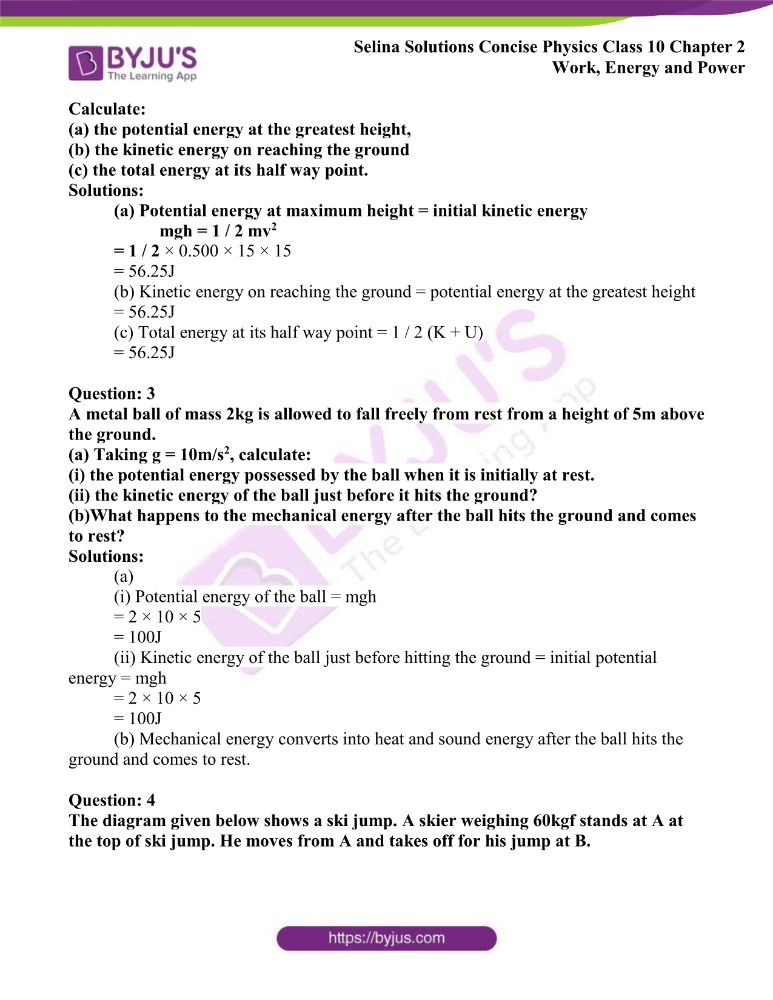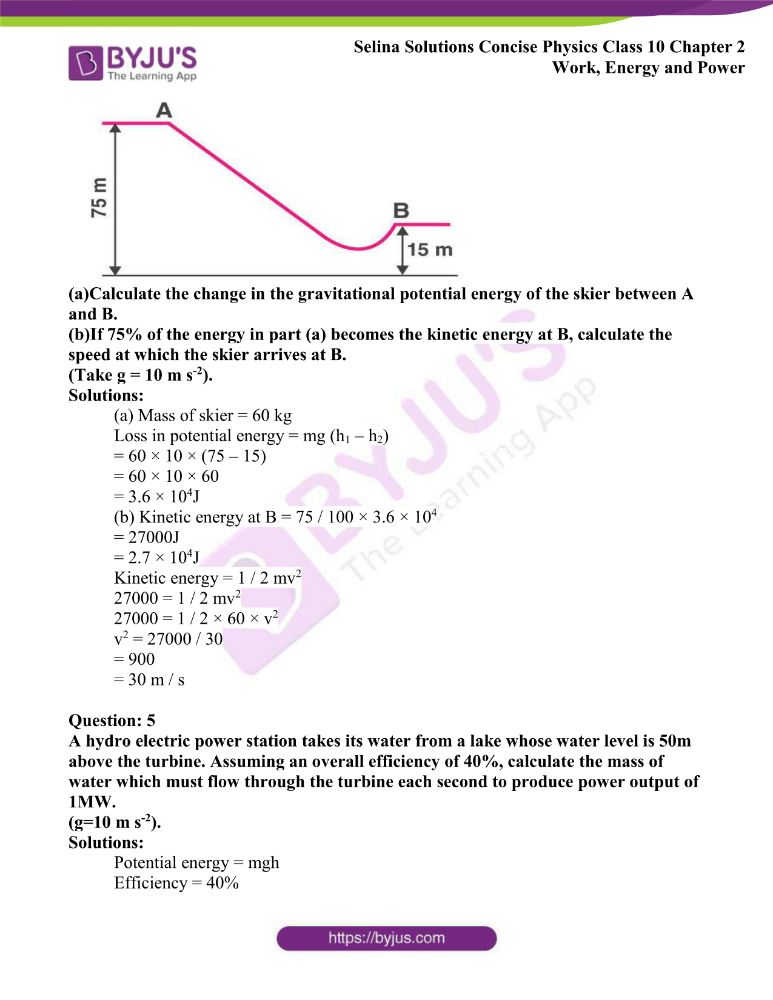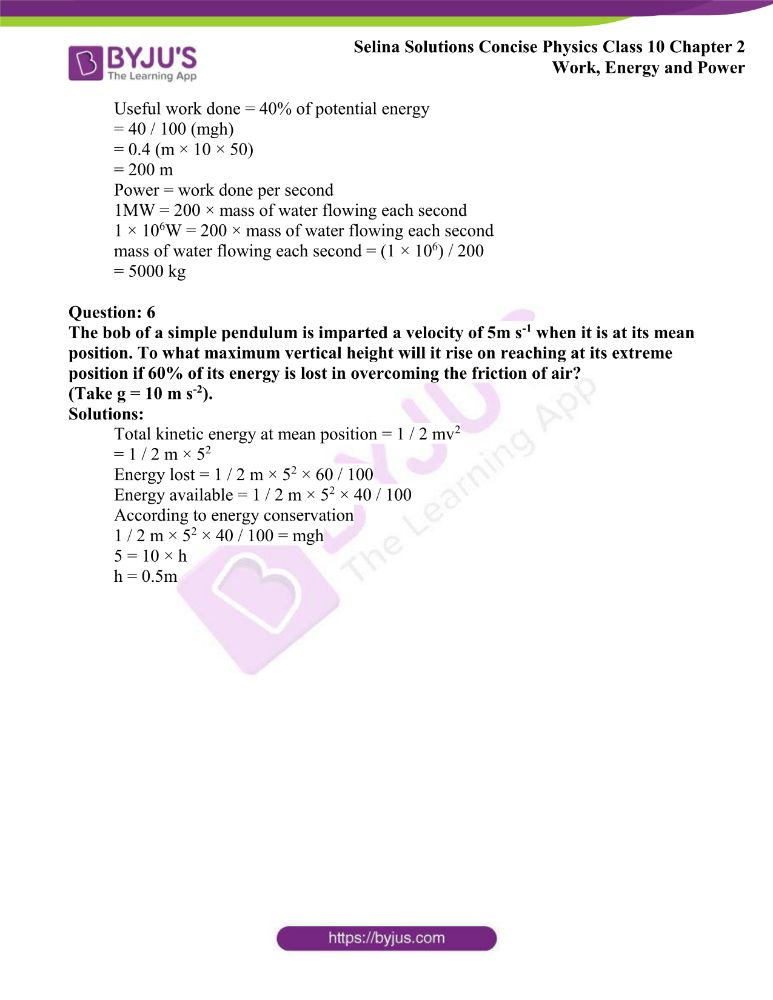### Access Answers of Physics Selina Solutions Concise Physics Class 10 Chapter 2 Work, Energy and Power

Exercise 2(A) page no: 27

Question: 1

Define work. When is work said to be done by a force?

Solutions:

When the force applied on a body makes the body move, then the work is said to be done. It is a scalar quantity.

Question: 2

How is the work done by a force measured when

(i) force is in direction of displacement,

(ii) force is at an angle to the direction of displacement?

Solutions:

(i) Work done when force is in direction of displacement,

W = F × S

(ii) Work done when force is at an angle to the direction of displacement,

W = F S cos θ

Question: 3

A force F acts on a body and displaces it by a distance S in a direction at an angle θ with the direction of force.

(a) Write the expression for the work done by the force.

(b) What should be the angle between force and displacement so that the work done is

(i) zero,

(ii) maximum?

Solutions:

(a) Work done when force is at an angle θ to the direction of displacement,

W = F S cos θ

(b) (i) The angle between force and displacement should be 900 as cos 900 = 0 for zero work done.

W = F S cos 900

= FS × 0

(ii) The angle between force and displacement should be 00 as cos 00 = 1 for maximum work done.

Then, W = FS cos 00

= FS

Question: 4

A body is acted upon by a force. State two conditions when the work done is zero.

Solutions:

When the work done is zero, the two conditions are:

(i) When there is no displacement,

S = 0 and

(ii) To the direction of force when the displacement is normal,

θ = 900

Question: 5

State the condition when the work done by a force is (i) positive, (ii) negative. Explain with the help of examples.

Solutions:

(i) The work done is positive, if the body’s displacement is in the direction of force,

Therefore W = F × S

Example: When the coolie raises the load against the force of gravity, then the work done by coolie on the load. Both the displacement and the force exerted by coolie are in upward direction.

(ii) The work done is negative, if the body’s displacement is in the direction opposite to the force,

Therefore W = – F × S

Example: The force of friction between the body and the surface is in a direction opposite to the motion of the body, when a body moves on a surface and hence work done by the force of friction is negative.

Question: 6

A body is moved in a direction opposite to the direction of force acting on it. State, whether the work is done by the force or work, is done against the force.

Solutions:

Work is done against the force when a body is moved in a direction opposite to the direction of force acting on it.

Question: 7

When a body moves in a circular path, how much work is done by the body? Give reason.

Solutions:

No work is done when a body moves in a circular path, since the force on the body is directed towards the centre of the circular path (the body is acted upon by the centripetal force), while at all instants the displacement is along the tangent to the circular path, i.e normal to the direction of the force.

Question: 8

A satellite revolves around the earth in a circular orbit. What is the work done by the satellite? Give reason.

Solutions:

As the force of gravity acting on the satellite is perpendicular to the displacement of the satellite, we get that,

Work done by the force of gravity is zero

Question: 9

State whether work is done or not by writing yes or no, in the following cases?

(a) A man pushes a wall.

(b) A coolie stands with a box on his head for 15 min.

(c) A boy climbs up 20 stairs.

Solutions:

(a) No, work is done by a man

(b) No, work is not done by a coolie

(c) Yes, work is done by a boy climbing up a stairs

Question: 10

A coolie X Carrying a load on his head climbs up a slope and another coolie Y carrying the identical load on his head move the same distance on a frictionless horizontal platform. Who does more work? Explain the reason.

Solutions:

The capacity to do work is energy and the energy spent is equal to the work done. The coolie X will do more work as the work done by him will involve a change in potential energy, kinetic energy and loss of energy due to friction whereas the work done by the coolie Y carrying the load in horizontal frictionless surface does not involve a change in potential energy and work done by the friction is also zero.

Question: 11

The work done by a fielder when he takes a catch in a cricket match, is negative. Explain.

Solutions:

The work done by a fielder is negative when he takes a catch because the force applied by the fielder is in the opposite direction of displacement of the ball.

Question: 12

Give an example when work done by the force of gravity acting on a body is zero even though the body gets displaced from its initial position.

Solutions:

The displacement is in the horizontal direction when a coolie carries a load walking on a ground while the force of gravity acts vertically downward. Hence the work done by the force of gravity is zero.

Question: 13

What are the S.I. and C.G.S. units of work? How are they related? Establish the relationship.

Solutions:

The S.I unit of work is Joule and C.G.S unit of work is erg

Relation between joule and erg:

1 joule = 1N × 1m

But 1 N = 105 dyne and 1 m = 102 cm

Therefore 1 joule = 105 dyne × 102 cm

= 107 dyne × cm

= 107 erg

∴1 joule = 107 erg

Question: 14

State and define the S.I. unit of work.

Solutions:

The S.I unit of work is Joule. When a force of 1 newton displaces a body through a distance of 1 metre in its direction then 1 joule of work is said to be done.

Question: 15

Express joule in terms of erg.

Solutions:

1 joule = 1N × 1m

But 1 N = 105 dyne and 1 m = 102 cm

Therefore 1 joule = 105 dyne × 102 cm

= 107 dyne × cm

= 107 erg

∴1 joule = 107 erg

Question: 16

A body of mass m falls through a height h. Obtain an expression for the work done by the force of gravity.

Solutions:

Let a body of mass m falls through a height h either vertically or inclined plane. For example: a hill, slope or staircase. The force of gravity on the body is F = mg acting vertically downwards and the vertical displacement in the direction of force is S = h. Therefore the work done by the force of gravity is

W = FS = mgh

Question: 17

A boy of mass m climbs up a stairs of vertical height h.

(a) What is the work done by the boy against the force of gravity?

(b) What would have been the work done if he uses a lift in climbing the same vertical height?

Solutions:

Let a boy of mass m climb up through a vertical height h either through a staircase or using a lift. F = mg is the force of gravity acting vertically downwards and the vertical displacement in the direction opposite to the force is

S = – h

Therefore the work done by the force of gravity on a boy is

W = FS = – mgh or

The work W = mgh is done by a boy against the force of gravity.

Question: 18

Define the term energy and state its S.I unit.

Solutions:

The term energy is defined as ‘the energy of a body is its capacity to do work’. The S.I unit of energy is Joule.

Question: 19

What physical quantity does electron volt (eV) measure? How is it related to the S.I. unit of that quantity?

Solutions:

The energy of atomic particles is measured in electron volt (eV)

1 eV = charge on an electron × 1 volt

= 1.6 × 10-19 coulomb × 1 volt

= 1.6 × 10-19 J

Question: 20

Complete the following sentence:

(a) 1 J = _____ calorie.

(b) 1 kWh = ______ J.

Solutions:

(a) 1 J = 0.24 calorie

(b) 1 kWh = 3.6 × 106 J

Question: 21

Name the physical quantity which is measured in calorie. How is it related to the S.I unit of that quantity?

Solutions:

Heat energy is measured in calorie.

1 calorie = 4.18 joule

Question: 22

Define a kilowatt-hour. How is it related to joule?

Solutions:

The energy spent (or work done) by a source of power 1 kW in 1 h is known as kilowatt-hour.

1 kWh = 3.6 × 106 J

Question: 23

Define the term power. State its S.I. unit.

Solutions:

The term power is defined as the rate of doing work. The S.I unit of power is the watt (W)

Question: 24

State two factors on which power spent by a source depends. Explain your answer with examples.

Solutions:

The power spent by a source depends on two factors

(i) The amount of work done by a source and

(ii) The time taken by the source to do the said work.

For example:

If a coolie A takes 1 minute to lift a load to the roof of a bus and a coolie B takes 2 minutes to lift a load to the roof of the same bus. The work done by both the coolies remain the same, but the power spent by the coolie A is twice the power spent by the coolie B because coolie A does the work faster than B.

Question: 25

Differentiate between work and power.

Solutions:

 Work Power Work done by a force is equal to the product of force and the displacement in the direction of the force. Power of a source is the rate of doing work by it. Work done does not depend on time Power spent depends on the time in which work is done. S.I unit of work is the joule (J) S.I unit of power is the watt (W)

Question: 26

Differentiate between energy and power.

Solutions:

 Energy Power The energy of a body is its capacity to do work. Power of a source is the rate at which energy is supplied by it Energy spent does not depend on time Power depends on the time in which energy is spent S.I unit of energy is joule (J) S.I unit of power is the watt (W)

Question: 27

State and define the S.I unit of power.

Solutions:

The S.I unit of power is the watt (W)

The power spent is said to be 1 watt, if 1 joule of work is done in 1 second.

Question: 28

(a) Name the physical quantity measured in terms of horsepower.

(b) How is horsepower related to the S. I. unit of power?

Solutions:

(a) The physical quantity measured in terms of horsepower is Power.

(b) It is related to the S.I unit watt as given below

1 horsepower = 746 watt

Question: 29

Differentiate between watt and watt-hour.

Solutions:

The unit of power is the watt (W) while the unit of work is watt-hour (Wh)

Since power × time = work or energy

Question: 30

Name the quantity which is measured in

(a) kWh

(b) kW

(c) Wh

(d) eV

Solutions:

(a) Energy is measured in kWh

(b) Power is measured in kW

(c) Energy is measured in Wh

(d) Energy is measured in eV

Question: 31

Is it possible that no transfer of energy takes place even when a force is applied to a body?

Solutions:

Yes, if the body is acted upon by the force normal to the displacement, there is no transfer of energy. The force is normal to the displacement when the body is moving in a circular path and the work done is zero. Hence, there is no transfer of energy.

MULTIPLE CHOICE TYPE

Question: 1

One horsepower is equal to:

a. 1000 W

b. 500 W

c. 764 W

d. 746 W

Solutions:

One horsepower is equal to (d) 746 W

Question: 2

kWh is the unit of:

a. Power

b. Force

c. Energy

d. None of these

Solutions:

kWh is the unit of (c) energy

NUMERICAL

Question: 1

A body, when acted upon by a force of 10 kgf, gets displaced by 0.5 m. Calculate the work done by the force, when the displacement is

(i) in the direction of force,

(ii) at an angle of 60o with the force, and

(iii) normal to the force. (g= 10Nkg-1)

Solutions:

Force acting on the body = 10 kgf

= 10 × 10 N

= 100 N

Displacement, S = 0.5 m

Work done = force × displacement in the direction of force

(i) W = F × S

W = 100 × 0.5

= 50 J

(ii) Work = force × displacement in the direction of force

W = F × S cos θ

W = 100 × 0.5 cos 600

W = 100 × 0.5 × 0.5 [cos 600 = 0.5]

W = 25 J

(iii) Normal to the force

Work = force × displacement in the direction of force

W = F × S cos θ

W = 100 × 0.5 cos 900

W = 100 × 0.5 × 0 [cos 900 = 0]

W = 0

Question: 2

A boy of mass 40kg climbs up the stairs and reaches the roof at a height 8m in 5 s. Calculate:

(i) The force of gravity acting on the boy,

(ii)The work done by him against gravity,

(iii)The power spent by the boy.

(take g= 10ms-2)

Solutions:

Given

Mass of a boy = 40 kg

Vertical height moved by a boy, h = 8 m

Time taken by a boy, t = 5 s

(i) Force of gravity acting on the boy

F = mg

= 40 × 10

= 400 N

(ii) Work done by a boy against the force of gravity while climbing,

Work done by a boy = Force × distance moved in the direction of force

W = F × S

W = 400 × 8

W = 3200 J

(iii) Power spent = (work done / time taken)

= 3200 / 5

= 640 W

Question: 3

A man spends 6.4 kJ energy in displacing a body by 64 m in the direction in which he applies force, in 2.5 s. Calculate: (i) the force applied and (ii) the power spent (in H.P) by the man.

Solutions:

Given

Work done by man = 6.4 kJ

Distance moved, S = 64 m

(i) Work done by the man = Force × distance moved in direction of force

Work, W = F × S

6.4 × 103 = F × 64

F = (6.4 × 103) / 64

F = 100 N

(ii) Power spent = (6.4 × 103) / 2.5

= 2560 W

1 H.P = 746 W

1 W = 1 / 746 H.P

2560 W = 2560 / 746 H.P

= 3.43 H.P

Question: 4

A weight lifter lifted a load of 200 kgf to a height of 2.5 m in 5 s. Calculate: (i) the work is done, and (ii) the power developed by him. Take g =10N/kg-1.

Solutions:

Force = mg

= 200 × 10

= 2000 N

Distance, S = 2.5 m

Time, t = 5 s

(i) Work done, W = FS

W = 2000 × 2.5 m

W = 5000 J

(ii) Power developed = (work done) / (time taken)

= 5000 J / 5 s

= 1000 W

Question: 5

A machine raises a load of 750N through a height of 16m in 5 s. Calculate:

(i) the energy spent by the machine.

(ii) the power of the machine if it is 100% efficient.

Solutions:

(i) Energy spent by machine or work done = FS

Work, W = 750 × 16

W = 12000 J

(ii) Power spent = work done / time taken

= 12000 J / 5s

= 2400 W

Question: 6

An electric heater of power 3 kW is used for 10 h. How much energy does it consume?

(i) kWh

(ii) joule.

Solutions:

Energy consumed = power × time

(i) Energy = 3 kW × 10 h

= 30 kWh

(ii) 1 kilowatt hour (kWh) = 3.6 × 106 J

30 kWh = 30 × 3.6 × 106 J

= 1.08 × 108 J

Question: 7

A water pump raises 50 litres of water through a height of 25m in 5 s. Calculate the power of the pump required.

(Take g= 10N kg-1 and density of water =1000kg m-3).

Solutions:

Volume of water = 50 L

= 50 × 10-3 m3

Density of water = 1000 kgm-3

Mass of water = Volume of water × density of water

= 50 × 10-3 × 1000

= 50 kg

Work done in raising 50 kg water to a height of 25 m against the force of gravity is

W = mg × h

W = mgh

Power P = (work done) / (time taken)

= mgh / t

= 50 × 10 × 25J / 5 s

= 2500W

Question: 8

A pump is used to lift 500kg of water from a depth of 80m in 10s. Calculate:

(a) The work done by the pump,

(b) The power at which the pump works, and

(c) The power rating of the pump if its efficiency is 40%. (Take g= 10m s-2).

Solutions:

Work done in raising a 500 kg mass to a height of 80 m against the force of gravity is:

(a) W = mg × h = mgh

W = 500 × 10 × 80

= 4 × 105 J

(b) Power at which pump works = work done / time taken

= mgh / t

= (500 × 10 × 80J) / 10 s

= (4 × 105) / 10

= 40 kW

(c) Efficiency = useful power / power input

Efficiency = 40 %

= 0.4

0.4 = 40 kW/ power input

Power input = 40 kW/0.4

= 100 kW

Question: 9

An ox can apply a maximum force of 1000N. It is taking part in a cart race and is able to pull the cart at a constant speed of 30m/s-1 while making its best effort. Calculate the power developed by the ox.

Solutions:

Given

Force = 1000 N

Velocity = 30 m/s

Power, P = force × velocity

P = 1000 × 30

= 30,000W

= 30 kW

Question: 10

The power of a motor is 40kW. At what speed can the motor raise a load of 20,000 N?

Solutions:

Given

Power = 40 kW

Force = 20,000 N

Power = force × velocity

Velocity = power / force

= 40 kW/ 20,000

= 40,000 / 20,000

= 2 m / s

= 2 ms-1

Question: 11

Rajan exerts a force of 150 N in pulling a cart at a constant speed of 10 m s-1. Calculate the power exerted.

Solutions:

Power exerted by Rajan due to force is

P = Fv

P = 150 × 10

P = 1500 W

Question: 12

A boy weighing 350 N climbs up 30 steps, each 20 cm high in 1 minute. Calculate:

(i) the work done, and

(ii) the power spent.

Solutions:

Total distance covered in 30 steps, S = 30 × 20 cm

= 600 cm

= 6 m

Work done by the boy in climbing = Force × distance moved in direction of force

Work, W = F × S

= 350 × 6

= 2100 J

Power developed = work done / time taken

= 2100 J / 60 s

= 35 W

Question: 13

It takes 20 s for a person A of mass 50 kg to climb up the stairs, while another person B of same mass does the same in 15 s. Compare the

(i) work done and

(ii) power developed by the persons A and B.

Solutions:

(i) The work done by two persons A and B is independent of time. Hence both will do the same amount of work. Hence,

Work done by A / work done by B

= 1 / 1

= 1: 1

(ii) The power developed by the persons A and B is calculated as shown below:

A takes 20 s to climb the stairs while B takes 15 s to do the same work. Hence, B does the work at a faster rate than A. Therefore more power is spent by B.

Power developed = 1 / time (amount of work done by A and B is same)

Power developed by A / Power developed by B = 15 / 20

= 3 / 4

= 3: 4

Question: 14

A boy of weight 40 kgf climbs up the 15 steps, each 15 cm high in 10 s and a girl of weight 20 kgf does the same in 5 s. Compare :

(i) the work done, and

(ii) the power developed by them. Take g = 10 N kg-1.

Solutions:

(i) Work done is

W = Fs = mgh

(Weight of boy) / (weight of girl) = 40 / 20

= 2 / 1

= 2: 1

(ii) Power developed is

Pboy / Pgirl = [(mboygh) / t] / [(mgirlgh) / t]

= (40 / 10) / (20 / 5)

Thus Pboy / Pgirl = (40 / 10) × (5 / 20)

= 1: 1

Question: 15

A man raises a box of mass 50kg to a height of 2m in 20s, while another man raises the same box to the same height in 50s.

(a) Compare: (i) the work done, and (ii) the power developed by them.

(b) Calculated: (i) the work done, and (ii) the power developed by each man. Take g = 10N kg-1.

Solutions:

(a) (i) Work done to raise the box of mass 50 kg is the same for both

(ii) Power = work done / time taken

The power taken by the first man is less. Hence powered developed is more.

(b) (i) Work done = 50 × 10 × 2

= 1000 J

(ii) Power developed by first man = 1000 / 20

= 50 W

Power developed by second man = 1000 / 50

= 20 W

Question: 16

A boy takes 3 minutes to lift a 20 litre water bucket from a 20 m deep well, while his father does it in 2 minutes. (a) Compare: (i) the work, and (ii) power developed by them. (b) How much work each does? Take density of water = 103 kg m-3 and g = 9.8 N kg-1.

Solutions:

(a) (i) Both the people carry same weight of water to the same height. So, work done is same for both. Hence, Work = 1: 1

(ii) Power developed is

Pboy / Pfather = [(W / tboy) / (W / tfather)]

= tfather / tboy

∴ Pboy / Pfather = 2 / 3

= 2: 3

(b) Mass of water lifted by both is

mw = p × V

= 103 kg / m3 × 20 × 10-3 m3

= 20 kg

So, the work done is

W = mwgh

= 20 × 9.8 × 20

W = 3920 J

W = 3.92 kJ

Exercise 2(B) page no: 40

Question: 1

What are the two forms of mechanical energy?

Solutions:

Kinetic energy and potential energy are the two forms of mechanical energy.

Question: 2

Name the form of energy which a wound-up watch spring possesses.

Solutions:

Wound up watch spring possesses an elastic potential energy.

Question: 3

Name the type of energy (kinetic energy K or potential energy U) possessed in the following cases:

(a) A moving cricket ball

(b) A compressed spring

(c) A moving bus

(d) A stretched wire

(e) An arrow shot out of a bow.

(f) A piece of stone placed on the roof.

Solutions:

(a) Kinetic energy (K)

(b) Potential energy (U)

(c) Kinetic energy (K)

(d) Potential energy (U)

(e) Kinetic energy (K)

(f) Potential energy (U)

Question: 4

Define the term potential energy of a body. Name its two forms and give one example of each.

Solutions:

Potential energy is defined as the energy possessed by a body at rest due to its position or size and shape is called the potential energy.

Different forms of potential energy are as follows:

(i) Gravitational potential energy: The potential energy possessed by a body due to the force of attraction of the earth on it, is called its gravitational potential energy.

Example: Due to its raised height, a stone at a height has gravitational potential energy.

(ii) Elastic potential energy: The potential energy possessed by a body in the deformed state due to change in its size and shape is called the elastic potential energy.

Example: Due to its compressed state, a compressed spring has elastic potential energy.

Question: 5

Name the form of energy which a body may possess even when it is not in motion. Give an example to support your answer.

Solutions:

The body possesses potential energy even when it is not in motion. For example: due to its raised position, a stone at a height has the gravitational potential energy.

Question: 6

What is meant by gravitational potential energy? Derive an expression for it for a body placed at a height above the ground.

Solutions:

Gravitational potential energy is defined as the potential energy possessed by a body due to the force of attraction of the earth on it, is called its gravitational potential energy.

The gravitational potential energy of a body placed at a height above the ground is measured by the work done in lifting it to that height against the force of gravity.

Let a body of mass m from the ground be lifted to a height h vertically. The force of gravity (mg) on the body acting vertically downwards must be equal to the least upward force F required to lift the body. The work done W on the body in lifting it to a height h is

W = force of gravity (mg) × displacement (h)

W = mgh

This work is stored in the body in the form of its gravitational potential energy when it is at a height h. Hence,

Gravitational potential energy U = mgh

Question: 7

Write an expression for the potential energy of a body of mass m placed at a height h above the earth’s surface. State the assumptions made, if any.

Solutions:

The work done on the body in lifting it to a height h is

W = force of gravity (mg) × displacement (h)

= mgh

Thus, this work is stored in the body in the form of its gravitational potential energy when it is at a height h.

Gravitational potential energy U = mgh

Question: 8

What do you understand by the kinetic energy of a body?

Solutions:

Kinetic energy is possessed by a body in motion. So, the energy possessed by a body due to its state of motion is called the kinetic energy.

Question: 9

(a) A body of mass m is moving with a velocity v. Write the expression for its kinetic energy.

(b) Show that the quantity 2K/v2 has the unit of mass, where K is the kinetic energy of the body.

Solutions:

(a) The expression for a body of mass m moving with a velocity v is given by

Kinetic energy = 1 / 2 × mass × (velocity)2

= 1 / 2 mv2

(b) 2K / v2 = Joules / (ms-1)2

= (kgms-2 × m) / m2s-2

= kg

Kg is the unit of mass

Question: 10

State the work–energy theorem.

Solutions:

According to the work theorem, the work done by a force in the same direction on a moving body is equal to the increase in its kinetic energy.

Question: 11

A body of mass m is moving with a uniform velocity u. A force is applied on the body due to which its velocity increases from u to v. How much work is being done by the force?

Solutions:

Let a body of mass m moving with an initial velocity u. When force is applied on the body along its direction of motion, an acceleration ‘a’ is produced and the velocity of the body changes from u to v in moving a distance S. So,

Work done by the force = force × displacement

W = F × S (1)

From relation v2 = u2 + 2aS

Displacement, S = (v2 – u2) / 2a

Force, F = ma

Substituting the values of F and S in equation (1), we get

W = F × S

W = ma × (v2 – u2) / 2a

= 1 / 2 mv2 – 1 / 2 mu2

W = kf – ki

Hence, work done on the body is equal to the increase in kinetic energy.

W = 1 / 2 m (v2 – u2)

Question: 12

A light mass and a heavy mass have equal momentum. Which will have more kinetic energy?

Solutions:

Kinetic energy and momentum are related as

K = p2 / 2m where p is momentum and k is kinetic energy

Both masses have the same momentum p. The kinetic energy k is inversely proportional to the mass of the body

Hence, a body of light mass has more kinetic energy because smaller the mass, larger is the kinetic energy.

Question: 13

Two bodies A and B of masses m and M (M≫ m) have same kinetic energy. Which body will have more momentum?

Solutions:

Kinetic energy is related to momentum and mass as

p = √2mk

Since the kinetic energy of both bodies are same, momentum is directly proportional to the square root of mass

Mass of body B is greater than that a body A

Therefore body B will have more momentum than body A.

Question: 14

Name the three forms of kinetic energy and give one example of each.

Solutions:

The three forms of kinetic energy are:

(i) Translational kinetic energy

Example: A car moving in a straight path.

(ii) Rotational kinetic energy

Example: A rotational wheel

(iii) Vibrational kinetic energy

Example: In a solid, the atoms vibrate about their mean positions.

Question: 15

State two differences between the potential energy and the kinetic energy.

Solutions:

 Potential energy Kinetic energy It can change only in the form of kinetic energy. It can change into any other form It does not depend on the speed of the body It depends on the speed of the body

Question: 16

Complete the following sentences:

(a)The kinetic energy of a body is the energy by virtue of its _______.

(b)The potential energy of a body is the energy by virtue of its _______.

Solutions:

(a) The kinetic energy of a body is the energy by virtue of its motion.

(b) The potential energy of a body is the energy by virtue of its position.

Question: 17

When an arrow is shot from a bow, it has kinetic energy in it. Explain briefly from where does it get its kinetic energy?

Solutions:

When the string of a bow is pulled, the work done is stored in the deformed state of the bow which is in the form of its elastic potential energy. When the string is released to shoot an arrow, the potential energy of the bow changes into the kinetic energy of the arrow which makes to move.

Question: 18

A ball is placed on a compressed spring. What form of energy does the spring possess? On releasing the spring, the ball flies away. Give a reason.

Solutions:

Due to the compressed state, the compressed spring has elastic potential energy. The potential energy of the spring changes into kinetic energy when it is released, which does work on the ball is placed on it and changes into kinetic energy of the ball due to which it flies away.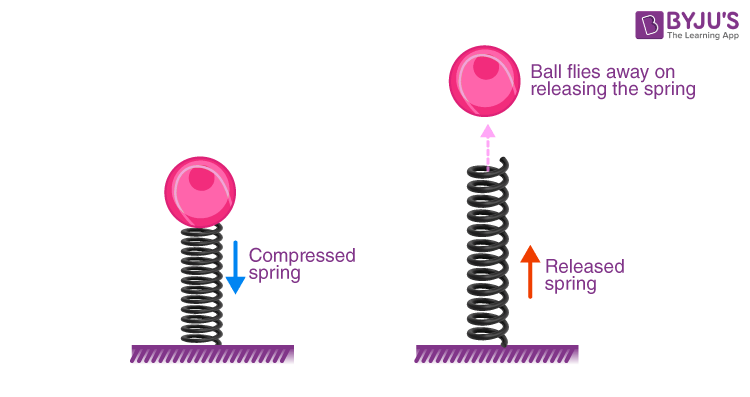Question: 19

A pebble is thrown up. It goes to a height and then comes back on the ground. State the different changes in the form of energy during its motion.

Solutions:

When the pebble is thrown upwards, the kinetic energy in it is converted to potential energy. Its kinetic energy is completely converted into potential energy at the top point in its motion. The potential energy is converted into kinetic energy while coming down and at the bottom, the potential energy is completely converted to kinetic energy.

Question: 20

In what way does the temperature of water at the bottom of a waterfall differ from the temperature at the top? Explain the reason.

Solutions:

During the fall of water from the height, the potential energy stored in water at a height changes into the kinetic energy. On striking the ground, a part of the kinetic energy of water changes into the heat energy which increases the temperature of the water.

Question: 21

Name the form of energy in which potential energy can change.

Solutions:

The potential energy can change only in the form of kinetic energy.

Question: 22

Name the form of mechanical energy, which is put to use.

Solutions:

Kinetic energy

Question: 23

Name six different forms of energy?

Solutions:

The six different forms of energy are

(i) Solar energy

(ii) Heat energy

(iii) Light energy

(iv) Hydro energy

(v) Nuclear energy

(vi) Mechanical energy

Question: 24

Energy can exist in several forms and may change from one form to another. For each of the following, state the energy changes that occur in:

(a) the unwinding of a watch spring

(b) a loaded truck when started and set in motion,

(c) a car going uphill,

(d) photosynthesis in green leaves,

(e) charging of a battery,

(f) respiration,

(g) burning of a match stick,

(h) explosion of crackers.

Solutions:

(a) Potential energy of a watch spring converts into kinetic energy

(b) Chemical energy of diesel or petrol converts into mechanical energy

(c) Kinetic energy converts into potential energy

(d) Light energy converts into chemical energy

(e) Electrical energy converts into chemical energy

(f) Chemical energy converts into heat energy

(g) Chemical energy converts into heat and light energy

(h) Chemical energy converts into heat, light and sound energy

Question: 25

State the energy changes in the following cases while in use:

(a)Loudspeaker

(b)A steam engine

(c)Microphone

(d)Washing machine

(e)A glowing electric bulb

(f)Burning coal

(g)A solar cell

(h)Biogas burner

(i)An electric cell in a circuit

(j)A petrol engine of a running car

(k)An electric iron

(l)A ceiling fan

(m)An electromagnet.

Solutions:

(a) Electrical energy changes into sound energy

(b) Heat energy changes into mechanical energy

(c) Sound energy changes into electrical energy

(d) Electrical energy changes into mechanical energy

(e) Electrical energy changes into light energy

(f) Chemical energy changes into heat energy

(g) Light energy changes into electrical energy

(h) Chemical energy changes into heat energy

(i) Chemical energy changes into electrical energy

(j) Chemical energy changes into mechanical energy

(k) Electrical energy changes into heat energy

(l) Electrical energy changes into mechanical energy

(m) Electrical energy changes into magnetic energy

Question: 26

Name the process used for producing electricity from nuclear energy.

Solutions:

The process used for producing electricity from nuclear energy is nuclear fission

Question: 27

Is it practically possible to convert a form of energy completely into the other useful form? Explain your answer.

Solutions:

No. Because, whenever there is a conversion of energy completely into another useful form, a part of the energy is dissipated in the form of heat which is lost to the surroundings.

Question: 28

Solutions:

During the transformation of energy from one form to another, a part of energy gets converted to some undesirable form or a part of it is lost to the surroundings due to the friction or radiations which cannot be used for productive purpose. This is known as dissipation of energy or degradation of energy.

Question: 29

What do you mean by degradation of energy? Explain it by taking one example of your daily life.

Solutions:

During the transformation of energy from one form to another, a part of energy gets converted to some undesirable form or a part of it is lost to the surroundings due to the friction or radiations which cannot be used for productive purpose. This is known as dissipation of energy or degradation of energy.

Example: During the glow of light bulb, a major part of the electrical energy utilised is converted to heat energy while some part is converted to useful light energy.

Question: 30

Complete the following sentence:

The conversion of part of energy into an undesirable form is called……………..

Solutions:

The conversion of part of energy into an undesirable form is called the degradation of energy.

MULTIPLE CHOICE TYPE

Question: 1

A body at a height possesses:

a. Kinetic energy

b. Potential energy

c. Solar energy

d. Heat energy

Solutions:

A body at a height possesses (b) potential energy.

Question: 2

In an electric cell while in use, the change in energy is from:

a. Electrical to mechanical

b. Electrical to chemical

c. Chemical to mechanical

d. Chemical to electrical

Solutions:

In an electric cell while in use, the change in energy is from chemical to electrical

NUMERICAL

Question: 1

Two bodies of equal masses are placed at heights h and 2h. Find the ratio of their gravitational potential energies.

Solutions:

Height H1 = h

Height H2 = 2h

Mass of body 1 = m

Mass of body 2 = m

Gravitational potential energy of body 1 = mgH1

= mgh

Gravitational potential energy of body 2 = mgH2

= mg(2h)

Ratio of gravitational potential energies = mgh / mg(2h)

= mgh / 2mgh

= 1 / 2

= 1: 2

Question: 2

Find the gravitational potential energy of 1kg mass kept at a height of 5m above the ground if g =10ms-2.

Solutions:

Given

Mass, m = 1 kg

Height, h = 5 m

Gravitational potential energy = mgh

= 1 × 10 × 5

= 50 J

Question: 3

A box of weight 150 kgf has gravitational potential energy stored in it equal to 14700 J. Find the height of the box above the ground.

Solutions:

Gravitational potential energy = 14700 J

Force of gravity = mg

= 150 × 9.8 N/kg

= 1470 N

Gravitational potential energy = mgh

14700 = 1470 × h

h = 10 m

Question: 4

A body of mass 5 kg falls from a height of 10 m to 4 m. Calculate:

(i) the loss in potential energy of the body,

(ii) the total energy possessed by the body at any instant? (Take g = 10 ms-2).

Solutions:

(i) Mass of the body, M = 5 kg

Potential energy at height 10 m = mgh

= 5 × 10 × 10

= 500 J

Potential energy at height 4 m = mgh

= 5 × 10 × 4

= 200 J

Loss in potential energy = (500 – 200) J

= 300 J

(ii) The total energy possessed by the body at any instant remains constant for free fall

It is equal to the sum of P.E and K.E

Thus, at height 10 m, K.E = 0

Therefore total energy = P.E + K.E

Total energy = 500 + 0

= 500 J

Question: 5

Calculate the height through which a body of mass 0.5 kg is lifted if the energy spent in doing so is 1.0 J. Take g = 10m/s-2.

Solutions:

Given

Mass = 0.5 kg

Energy = 1 J

Gravitational potential energy = mgh

1 = 0.5 × 10 × h

1 = 5h

h = 1 / 5

h = 0.2 m

Question: 6

A boy weighing 25 kgf climbs up from the first floor at a height of 3 m above the ground to the third floor at a height of 9 m above the ground. What will be the increase in his gravitational potential energy?

(Take g=10 N kg-1).

Solutions:

Force of gravity on boy = mg

= 25 × 10

= 250 N

Increase in gravitational potential energy = Mg (h2 – h1)

= 250 × (9 – 3)

= 250 × 6

=1500 J

Question: 7

A vessel containing 50 kg of water is placed at a height 15m above the ground. Assuming the gravitational potential energy at the ground to be zero, what will be the gravitational potential energy of water in the vessel? (g = 10ms-2).

Solutions:

Given

Mass of water, m = 50 kg

Height, h = 15 m

Gravitational potential energy = mgh

= 50 × 10 × 15

= 7500 J

Question: 8

A man of mass 50 kg climbs up a ladder of height 10m. Calculate: (i) the work done by the man, (ii) the increase in his potential energy.

(g= 9.8m s-2).

Solutions:

Given

Mass of man = 50 kg

Height of ladder, h2 = 10 m

(i) Work done by man = mgh2

= 50 × 9.8 × 10

= 4900 J

(ii) Increase in his potential energy

Height, h2 = 10 m

Reference point is ground, h1 = 0 m

Gravitational potential energy = Mg (h2 – h1)

= 50 × 9.8 × (10 – 0)

= 50 × 9.8 × 10

= 4900 J

Question: 9

A block A, whose weight is 100N, is pulled up a slope of length 5m by means of a constant force F (=100N) as illustrated.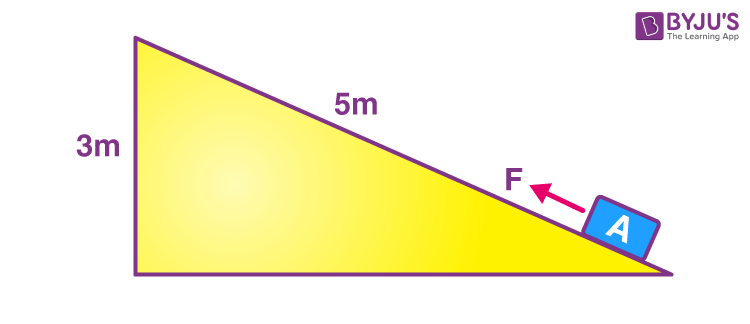(a) What is the work done by the force F in moving the block A, 5m along the slope?
(b) What is the increase in potential energy of the block A?
(c) Account for the difference in the work done by the force and the increase in potential energy of the block.

Solutions:

F = 100 N

Work done by the force in moving the block 5m along the slope = Force × displacement in the direction of the force

= 100 × 5

= 500 J

The potential energy gained by the block U = mgh (where h = 3m)

= 100 × 3

= 300 J

– is the potential energy gained by the block

The difference i.e 200 J energy is used in doing work against the force of friction between the block and the slope, which will appear as heat energy.

Question: 10

Find the kinetic energy of a body of mass 1kg moving with a uniform velocity of 10m s-1.

Solutions:

Mass, m = 1 kg

Velocity, v = 10 m / s

Kinetic energy = 1 / 2 × mass × (velocity)2

= 1 / 2 × 1 × (10)2

= 1 / 2 × 1 × 100

= 50 J

Questions: 11

If the speed of a car is halved, how does its kinetic energy change?

Solutions:

The kinetic energy decreases, if the speed is halved keeping the mass same. Since kinetic energy is proportional to the square of velocity, it becomes one-fourth.

Question: 12

Calculate the decrease in the kinetic energy of a moving body if its velocity reduces to half of the initial velocity.

Solutions:

Kinetic energy is directly proportional to the square of velocity.

Hence, velocity is reduced is half its original value. We get,

ΔK = K – Knew = 1 / 2 mv2 – 1 / 2 m (1 / 2 v)2

Hence, ΔK = 1 / 2 mv2 – 1 / 2 mv2 (1 / 4)

= 1 / 2 mv2 – 1 / 8 mv2

= 3 / 8 mv2

= 3 / 4 × 1 / 2 mv2

= 3 / 4K

Question: 13

Two bodies of equal masses are moving with uniform velocities v and 2v. Find the ratio of their kinetic energies.

Solutions:

Given

Velocity of first body v1 = v

Velocity of second body, v2 = 2v

Masses are same, so kinetic energy is directly proportional to the square of the velocity (K α v2)

Hence, ratio of their kinetic energies is

K1 / K2 = (v1)2 / (v2)2

= v2 / (2v)2

= v2 / 4v2

= 1 / 4

= 1: 4

Question: 14

A car is running at a speed of 15 km h-1 while another similar car is moving at a speed of 45 km h-1. Find the ratio of their kinetic energies.

Solutions:

KE = mv2

KE α v2

KE1 / KE2 = v12 / v22

KE1 / KE2 = (15 / 45)2

KE1 / KE2 = (1 / 3)2

KE1 / KE2 = 1 / 9

KE1 / KE2 = 1: 9

Question: 15

A ball of mass 0.5 kg slows down from a speed of 5m/s-1 to that of 3m/s-1. Calculate the change in kinetic energy of the ball.

Solutions:

Given

Mass of ball = 0.5 kg

Initial velocity = 5 m / s

Initial kinetic energy = 1 / 2 × mass × (velocity)2

= 1 / 2 × 0.5 × (5)2

= 1 / 2 × 0.5 × 25

= 6.25 J

Final velocity of the ball = 3 m / s

Final kinetic energy of the ball = 1 / 2 × mass × (velocity)2

= 1 / 2 × mass × (velocity)2

= 1 / 2 × 0.5 × (3)2

= 1 / 2 × 0.5 × 9

= 2.25J

So, change in the kinetic energy of the ball = 2.25 J – 6.25 J

= – 4J

Hence, there is a decrease in the kinetic energy of the ball.

Question: 16

A canon ball of mass 500g is fired with a speed of 15m/s-1. Find:

(i) its kinetic energy and

(ii) its momentum.

Solutions:

Given

A mass of cannon ball = 500 g

= 0.5 kg

Speed, v = 15 m / s

(a) Kinetic energy of ball = 1 / 2 × mass × (velocity)2

= 1 / 2× 0.5 × (15)2

= 1 / 2 × 0.5 × 225

= 56.25J

(b) Momentum of the ball = mass × velocity

= 0.5 × 15

= 7.5 kgm/s

Question: 17

A body of mass 10 kg is moving with a velocity 20m s-1. If the mass of the body is doubled and its velocity is halved, find: (i) the initial kinetic energy, and (ii) the final kinetic energy.

Solutions:

Let initial Mass, m1 = 10 kg and

Velocity, v1 = 20 m / s

Final mass, m2 = 2 × 10 kg and

Velocity, v2 = 20 / 2

= 10 m / s

Initial kinetic energy, K1 = 1 / 2 × mass × (velocity)2

= 1 / 2 × 10 × (20)2

= 1 / 2 × 10 × 20 × 20

= 2000J

Final kinetic energy, K2 = 1 / 2 × mass × (velocity)2

= 1 / 2 × 20 × (10)2

= 1 / 2 × 20 × 10 × 10

= 1000J

Therefore K1 / K2 = 2000 / 1000

= 2 / 1

= 2: 1

Question: 18

A truck weighing 1000 kgf changes its speed from 36 km/h-1 to 72 km/h-1 in 2 minutes. Calculate:

(i) the work done by the engine and

(ii) its power.

(g =10 m/s-2)

Solutions:

u = 36 km / h = 36 × 1000m / 3600s

= 10 m / s and

v = 72 km / h = 72 × 1000m / 3600s

= 20 m / s

Given

Mass of truck = 1000 kg

(i)

W = 1 / 2 × 1000 × (202 – 102)

W = 500 × (400 – 100)

W = 500 × 300

W = 150000J

W = 1.5 × 105J

(ii)

Power = work done / time taken

= 1.5 × 105 J / 120s

= 1.25 × 103 W

Question: 19

A body of mass 60 kg has the momentum 3000 kgm/s-1. Calculate:

(i) the kinetic energy and

(ii) the speed of the body.

Solutions:

Given

Mass of body = 60 kg

Momentum, p = 3000 kgm / s

(a) Kinetic energy = p2 / 2m

= (3000)2 / 2 × 60

= (3000 × 3000) / 120

= 75000J

= 7.5 × 104J

(b) Momentum = mass × velocity

3000 = 60 × velocity

Velocity = 3000 / 60

Velocity = 50 m / s

Question: 20

How much work is needed to be done on a ball of mass 50g to give it a momentum of 5 kg m s-1?

Solutions:

Momentum, p = 500 gcm / s

= 0.005 kgm / s

Mass of ball = 50 g

= 0.05 kg

Kinetic energy of the ball = p2 / 2m

= (0.005)2 / 2 × 0.05

= 250J

Question: 21

How much energy is gained by a box of mass 20 kg when a man

(a) carrying the box waits for 5 minutes for a bus?

(b) runs carrying the box with a speed of 3 m/s-1 to catch the bus?

(c) raises the box by 0.5 m in order to place it inside the bus? (g=10 m/s-2)

Solutions:

Given

Mass of box = 20 kg

(a) As there is no displacement of the man, so zero work is done

(b) Work done, Kinetic energy of man = 1 / 2 × mass × (velocity)2

= 1 / 2 × 20 × (3)2

= 1 / 2 × 20 × 9

= 90J

(c) Work done in raising the box, Potential energy = mgh

U = 20 × 10 × 0.5

= 100J

Question: 22

A bullet of mass 50g is moving with a velocity of 500m/s-1. It penetrates 10 cm into a still target and comes to rest. Calculate:

(a) the kinetic energy possessed by the bullet, and

(b) the average retarding force offered by the target.

Solutions:

Given

Mass of bullet = 50 g

= 0.05 kg

Velocity = 500 m / s

Distance penetrated by the bullet = 10 cm

= 0.1 m

(a) The kinetic energy of the bullet = 1 / 2 × mass × (velocity)2

= 1 / 2 × 0.05 × (500)2

= 1 / 2 × 0.05 × 500 × 500

= 6250J

(b) Work done by the bullet against the material of the target = resistance force × distance

6250 = resistance force × 0.1 m

Resistance force = 62500N

Question: 23

A spring is kept compressed by a small trolley of mass 0.5 kg lying on a smooth horizontal surface as shown. When the trolley is released, it is found to move at a speed of v = 2 m/s-1. What potential energy did the spring possess when compressed?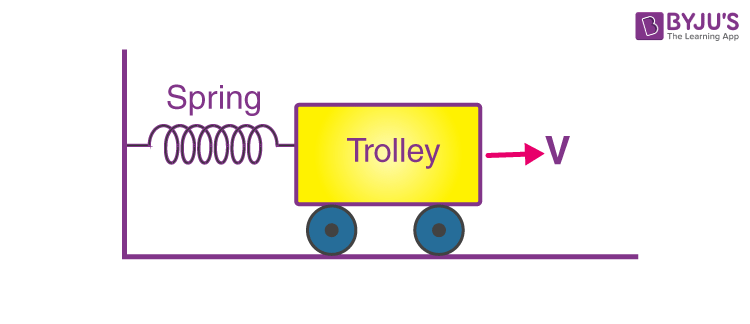Solutions:

Given

Mass of trolley = 0.5 kg

Velocity = 2 m / s

When the compressed spring is released, its potential energy is converted into kinetic energy completely.

Potential energy of compressed spring = kinetic energy of moving trolley

Kinetic energy of trolley = 1 / 2 × mass × (velocity)2

= 1 / 2 × 0.5 × (2)2

= 1 / 2 × 0.5 × 2 × 2

= 1J

So, potential energy of compressed spring = 1.0J

Exercise 2(c) page n0: 46

Question: 1

State the Principle of conservation of energy.

Solutions:

According to the principle of conservation of energy, energy can neither be created nor can it be destroyed. It only changes from one form to the other.

Question: 2

What do you understand by the conservation of mechanical energy? State the condition under which the mechanical energy is conserved.

Solutions:

The law of conservation of mechanical energy states that, whenever there is an interchange between the potential energy and kinetic energy, the total mechanical energy remains constant i.e K + U = constant when there are no frictional forces.

When there are no frictional forces for a given system only then the mechanical energy is conserved i.e between body and air. Hence, conservation of mechanical energy is strictly valid only in vacuum, where friction due to air is absent.

Question: 3

Name two examples in which the mechanical energy of a system remains constant.

Solutions:

The two examples in which the mechanical energy of a system remains constant are the motion of a simple pendulum and motion of a freely falling body.

Question: 4

A body is thrown vertically upwards. Its velocity keeps on decreasing. What happens to its kinetic energy as its velocity becomes zero?

Solutions:

When a body is thrown vertically upwards, its kinetic energy changes into potential energy and its velocity becomes zero.

Question: 5

A body falls freely under gravity from rest. Name the kind of energy it will possess

(a)At the point from where it falls.

(b)While falling

(c)On reaching the ground.

Solutions:

(a) An energy possessed by the body at the point from where it falls is potential energy.

(b) The energy possessed by the body while falling is potential energy and kinetic energy.

(c) The energy possessed by the body on reaching the ground is kinetic.

Question: 6

Show that the sum of kinetic energy and potential energy (i.e., total mechanical energy) is always conserved in the case of a freely falling body under gravity (with air resistance neglected) from a height h by finding it when

(i) the body is at the top,

(ii) the body has fallen a distance x,

(iii) the body has reached the ground.

Solutions:

Let a body of mass m be falling freely under gravity from a height h above the ground (i.e from position A). Now calculate sum of kinetic energy K and potential energy U at various positions, say at A (at height h above the ground), at B (when it has fallen through a distance x) and at C (on the ground).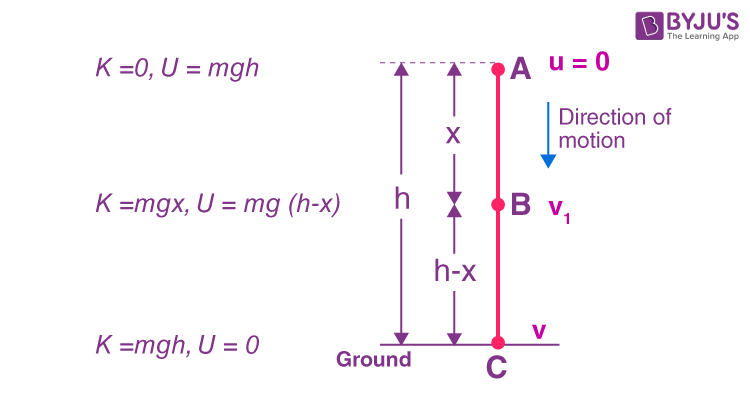(i) At the position A (at height h above the ground):

Initial velocity of body = 0 (since body is at rest at A)

Therefore Kinetic energy K = 0

Potential energy U = mgh

Hence total energy = K + U

= 0 + mgh

= mgh (1)

(ii) At the position B (when it has fallen a distance x):

Let v1 be the velocity acquired by the body at B after falling through a distance x. Then u = 0, s = x, a = g

From equation v2 = u2 + 2aS

V12 = 0 + 2gx

= 2gx

∴ Kinetic energy K = 1 / 2 mv12

= 1 / 2 m (2gx)

= mgx

Now at B, height of body above the ground = h – x

∴ Potential energy U = mg (h – x)

Hence, total energy = K + U

= mgx + mg (h – x)

= mgh (2)

(iii) At position C (on the ground):

Let the velocity acquired by the body on reaching the ground be v. Then u = 0, s = h, a = g

From equation: v2 = u2 + 2aS

v2 = 02 + 2gh

v2 = 2gh

or Kinetic energy K = 1 / 2 mv2

= 1 / 2 m (2gh)

= mgh

And potential energy U = 0 (at the ground when h = 0)

So, total energy = K + U

= mgh + 0

= mgh (3)

Thus from equation (1), (2) and (3) we note that the total mechanical energy i.e the sum of kinetic energy and potential energy always remain constant at each point of motion and it is equal to the initial potential energy at height h.

Question: 7

A pendulum is oscillating on either side of its rest position. Explain the energy changes that take place in the oscillating pendulum. How does the mechanical energy remain constant in it? Draw necessary diagram.

Solutions:

The kinetic energy decreases and the potential energy becomes maximum at B where it is momentarily at rest, when the bob swings from A to B.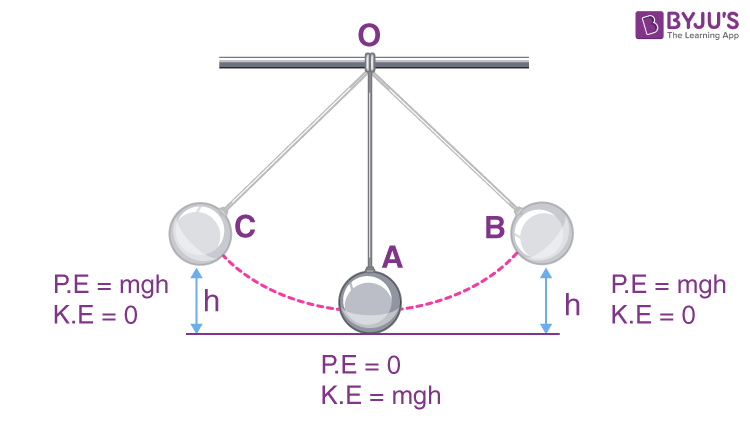From B to A, again the potential energy changes into kinetic energy and this process repeat again and again. So, while swinging, the bob has the potential energy at the extreme position B or C and kinetic energy at resting position A. The bob has both the kinetic energy and potential energy at an intermediate position (between A and B or between A and C) and the sum of both the energies (i.e the total mechanical energy) remains constant throughout the swing.

Question: 8

A pendulum with a bob of mass m is oscillating on either side from its resting position A between the extremes B and C at a vertical height h above A. What is the kinetic energy K and potential energy U when the pendulum is at positions

(i) A,

(ii) B and

(iii) C?

Solutions:

(i) At position A: pendulum has maximum kinetic energy and its potential energy is zero at its resting position. So, K = mgh and U = 0

(ii) At position B: The kinetic energy decreases and the potential energy increases. So, K = 0 and U = mgh

(iii) At position C: kinetic energy K = 0 and potential energy U = mgh.

Question: 9

Name the type of energy possessed by the bob of a simple pendulum when it is at

(a) the extreme position,

(b) the mean position, and

(c) between the mean and extreme positions.

Solutions:

(a) Energy possessed by the bob of a simple pendulum at extreme position is potential energy

(b) Energy possessed by the bob of a simple pendulum at mean position is kinetic energy

(c) Energy possessed by the bob of a simple pendulum between mean and extreme position are both kinetic energy and potential energy.

MULTIPLE CHOICE TYPE

Question: 1

A ball of mass m is thrown vertically up with an initial velocity so as to reach a height h. The correct statement is:

a. Potential energy of the ball at the ground is mgh.

b. Kinetic energy to the ball at the ground is zero.

c. Kinetic energy of the ball at the highest point is mgh.

d. The potential energy of the ball at the highest point is mgh.

Solutions:

The correct statement is ‘potential energy of the ball at the highest point is mgh.

Question: 2

A pendulum is oscillating on either side of its rest position. The correct statement is :

(a) It has only the kinetic energy at its each position.

(b) It has the maximum kinetic energy at its extreme position.

(c) It has the maximum potential energy at its mean position.

(d) The sum of its kinetic and potential energy remains constant throughout the motion.

Solutions:

The correct statement is ‘the sum of its kinetic and potential energy remains constant throughout the motion.

NUMERICAL

Question: 1

A ball of mass 0.20 kg is thrown vertically upwards with an initial velocity of 20m/s-1 Calculate the maximum potential energy it gains as it goes up.

Solutions:

Potential energy at the maximum height = initial kinetic energy

= 1 / 2 mv2

= 1 / 2 × 0.20 × 20 × 20

= 40J

Question: 2

A stone of mass 500 g is thrown vertically upwards with a velocity of 15m/s-1. Calculate:

(a) the potential energy at the greatest height,

(b) the kinetic energy on reaching the ground

(c) the total energy at its half waypoint.

Solutions:

(a) Potential energy at maximum height = initial kinetic energy

mgh = 1 / 2 mv2

= 1 / 2 × 0.500 × 15 × 15

= 56.25J

(b) Kinetic energy on reaching the ground = potential energy at the greatest height

= 56.25J

(c) Total energy at its halfway point = 1 / 2 (K + U)

= 56.25J

Question: 3

A metal ball of mass 2kg is allowed to fall freely from rest from a height of 5m above the ground.

(a) Taking g = 10m/s2, calculate:

(i) the potential energy possessed by the ball when it is initially at rest.

(ii) the kinetic energy of the ball just before it hits the ground?

(b)What happens to the mechanical energy after the ball hits the ground and comes to rest?

Solutions:

(a)

(i) Potential energy of the ball = mgh

= 2 × 10 × 5

= 100J

(ii) The kinetic energy of the ball just before hitting the ground = initial potential energy = mgh

= 2 × 10 × 5

= 100J

(b) Mechanical energy converts into heat and sound energy after the ball hits the ground and comes to rest.

Question: 4

The diagram given below shows a ski jump. A skier weighing 60kg stands at A at the top of ski jump. He moves from A and takes off for his jump at B.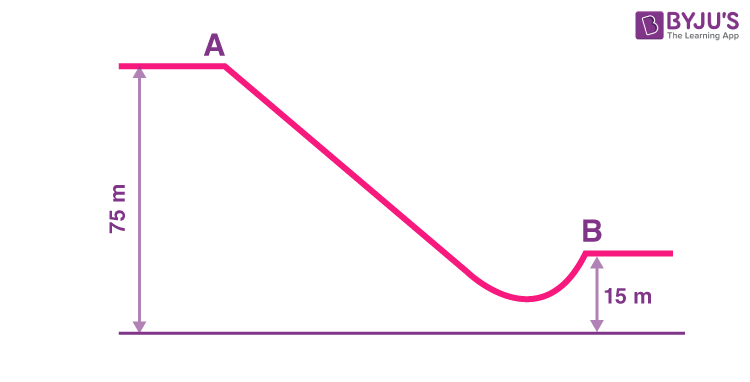(a)Calculate the change in the gravitational potential energy of the skier between A and B.

(b)If 75% of the energy in part (a) becomes the kinetic energy at B, calculate the speed at which the skier arrives at B.

(Take g = 10 m s-2).

Solutions:

(a) Mass of skier = 60 kg

Loss in potential energy = mg (h1 – h2)

= 60 × 10 × (75 – 15)

= 60 × 10 × 60

= 3.6 × 104J

(b) Kinetic energy at B = 75 / 100 × 3.6 × 104

= 27000J

= 2.7 × 104J

Kinetic energy = 1 / 2 mv2

27000 = 1 / 2 mv2

27000 = 1 / 2 × 60 × v2

v2 = 27000 / 30

= 900

= 30 m / s

Question: 5

A hydro electric power station takes its water from a lake whose water level is 50m above the turbine. Assuming an overall efficiency of 40%, calculate the mass of water which must flow through the turbine each second to produce power output of 1MW.

(g=10 m s-2).

Solutions:

Potential energy = mgh

Efficiency = 40%

Useful work done = 40% of potential energy

= 40 / 100 (mgh)

= 0.4 (m × 10 × 50)

= 200 m

Power = work done per second

1MW = 200 × mass of water flowing each second

1 × 106W = 200 × mass of water flowing each second

mass of water flowing each second = (1 × 106) / 200

= 5000 kg

Question: 6

The bob of a simple pendulum is imparted a velocity of 5m s-1 when it is at its mean position. To what maximum vertical height will it rise on reaching at its extreme position if 60% of its energy is lost in overcoming the friction of air?

(Take g = 10 m s-2).

Solutions:

Total kinetic energy at mean position = 1 / 2 mv2

= 1 / 2 m × 52

Energy lost = 1 / 2 m × 52 × 60 / 100

Energy available = 1 / 2 m × 52 × 40 / 100

According to energy conservation

1 / 2 m × 52 × 40 / 100 = mgh

5 = 10 × h

h = 0.5m

## Selina Solutions Concise Physics Class 10 Chapter 2 Work, Energy and Power

ICSE Selina Solutions Concise Physics Class 10 Chapter 2 Work, Energy and Power covers important topics namely work, measurement of work, work done by the force of gravity, units of work, power, units of power, units of energy, mechanical energy and its different forms, conservation of energy. These solutions also offer numerical problems, which help the students to understand the chapter in-depth. Students are advised to use these solutions PDF as a major study tool in clearing their doubts about the chapter. Solutions also help them to become experts in the subject.

List of subtopics covered in Selina Solutions Concise Physics Class 10 Chapter 2 Work, Energy and Power

 Number Subtopic 2.1 Work 2.2 Measurement of work 2.3 Work done by the force of gravity 2.4 Units of Work 2.5 Power 2.6 Units of Power 2.7 Energy 2.8 Units of Energy 2.9 Mechanical Energy and its different forms 2.10 Potential Energy 2.11 Gravitational Potential Energy 2.12 Kinetic Energy 2.13 Conservation of Potential Energy 2.14 Different forms of Energy 2.15 Conversion of one form of energy into the other form 2.16 Principle of conservation of Energy 2.17 Application of the principle of conservation of energy to a simple pendulum

List of Exercises

 Name of the exercise Page number 2(A) 27 2(B) 40 2(C) 46

### Key Features of Selina Solutions Concise Physics Class 10 Chapter 2 Work, Energy and Power

• Selina Solutions are comprehensive study materials
• Solutions are available step by step, which help in clearing students’ doubts
• Practising these solutions improves students performance in exams
• Solutions are well structured by BYJU’S faculty

The given solutions are as per the 2019-20 Concise Selina textbook. The Selina Solutions for the academic year 2023-24 will be updated soon.